Lately, along to malware analisys activity I started to study/test Windows to understand something more of its internals. The CVE here analyzed, has been a good opportunity to play with RPC and learn new funny things I never touched before and moreover it looked challenging enough to spent time.

This blogpost shows my roadmap to understand and reproduce the vulnerability, the analisys has the purpose just to arrive to a PoC of the vulnerability not to understand every bit of the RPC implementation neither to write an exploit for it.
RPC, for my basic knowlege, was just a way to call procedure remotely, e.g. client wants to execute a procedure in a server and get the result just like a syscall between user space and server space. This method allows in SW design to decouple goals and purpose and often lead to a good segregation of the permission. Despite this highly level, the RPC represented just another attack vector. The RPC protocol is implemented on top of various medium or transport protocols, e.g. pipes, UDP, TCP.
The vulnerability, as reported by Microsoft is an RCE on Remote Procedure Call. It’s not specified where is the bug, neither how to trigger it, so it is required to obtain the patch and diff it with a vulnerable version of the main library that implements the RPC, i.e. rpcrt4.dll.

Below is shown the exactly things I did to arrive to the vulnerable point, so the blogpost is more about finding a way without having complete knowledge of the analyzed object. Indeed the information I got about the RPC internals are shown here in a logic-time order so they are not represented in a structured way, as is usually done at the end of an analisys.

## Search for the vulnerability

Before starting with the usual binary diff, it’s required to get the right windows version in order to get the vulnerble library. I was lucky, my FLARE mount Windows 10 version 21H2 that is vulnerable according to the cpe published by the NIST. Before patching the systems it’s required to download the windows symbols (PDB) just to have the Windows symbols in Ghidra.

The symbols could be downloaded with the following commands, it’s required to have installed windows kits:

``````    cd "C:\Program Files (x86)\Windows Kits\10\Debuggers\x64\"
``````

After, downloaded the symbols I opened the library rpcrt4.dll, that is supposed to be vulnerable, in Ghidra then resolved symbols loading the right PDB and exported the Binary for Bindiff.

The hash of my rpcrt4.dll library is: b35fdb8d452e39cdf4393c09530837eff01d33c7

Since my windows version is: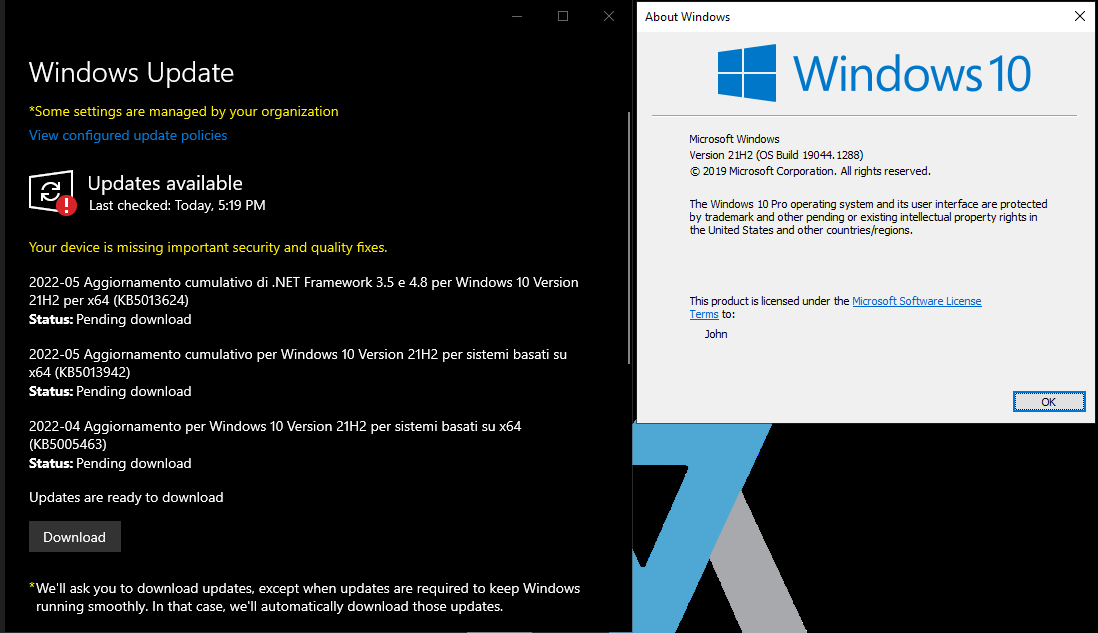The patch to download, obtained from Microsoft, is the following: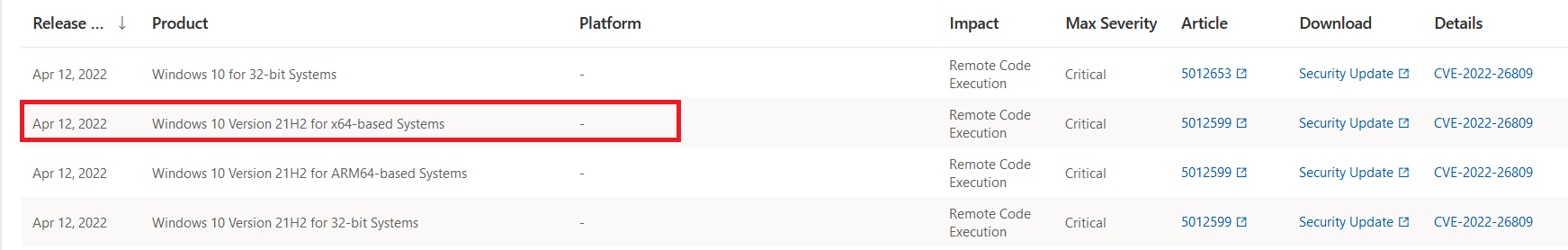After applying the patch the system contains a new rpcrt4.dll having SHA1: d78a9d416a1187da8550fb0d5a4bace48cfa8179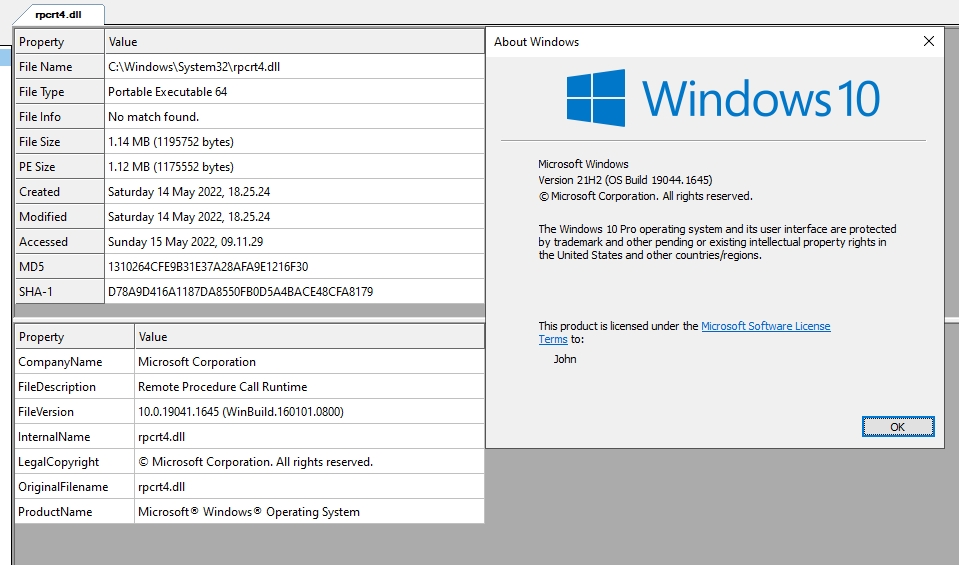### Bindiff

In order to load into ghidra the patched library has been necessary to download again the symbols and import the new PDB. After this operation, the binaries, i.e. the vulnerable and the patched one, are exported for bindiff and a binary diff is executed on them.

In the following image it’s clear that the patch introduced new functions and some other are not available anymore.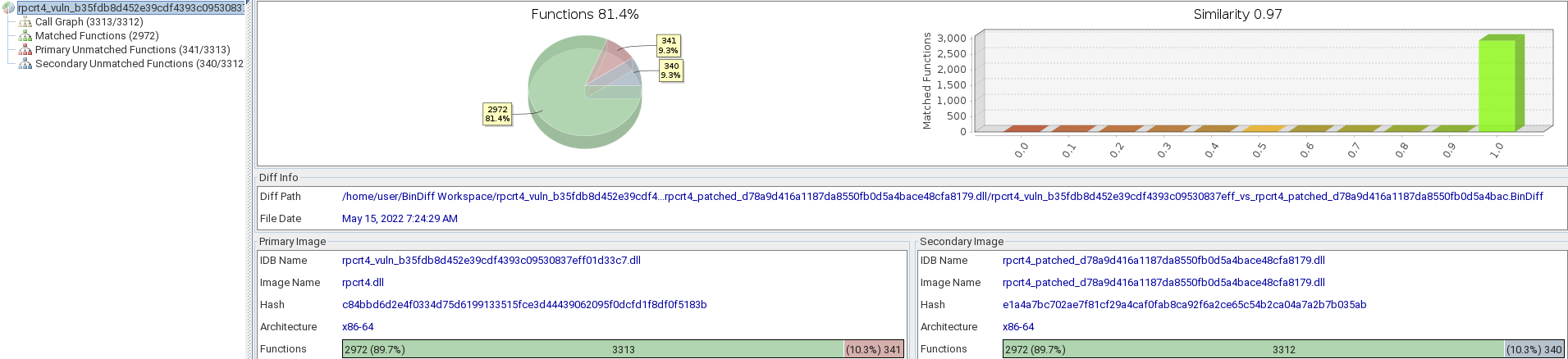Indeed, only 97% of similarity with ~640 unmatched functions. Since I am not an expert of the RPC internals, I started investigate the differencies starting from the functions that have an high percent of similarity tending to 100%.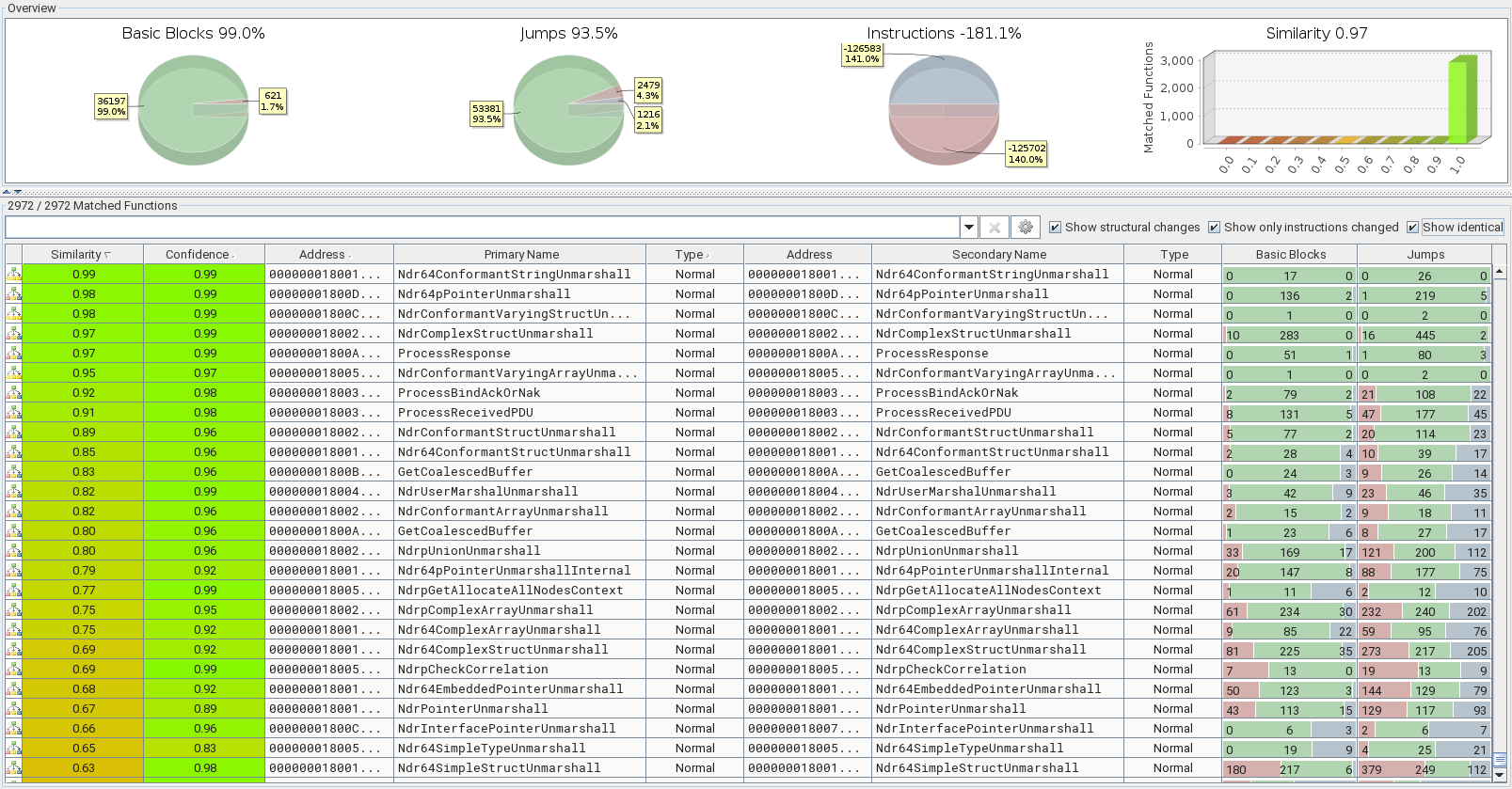I was lucky, the fix seems to be in the ProcessReceivedPDU() routine, indeed seems that in the new rpcrt4.dll version has been added a function to check the sum between two elements.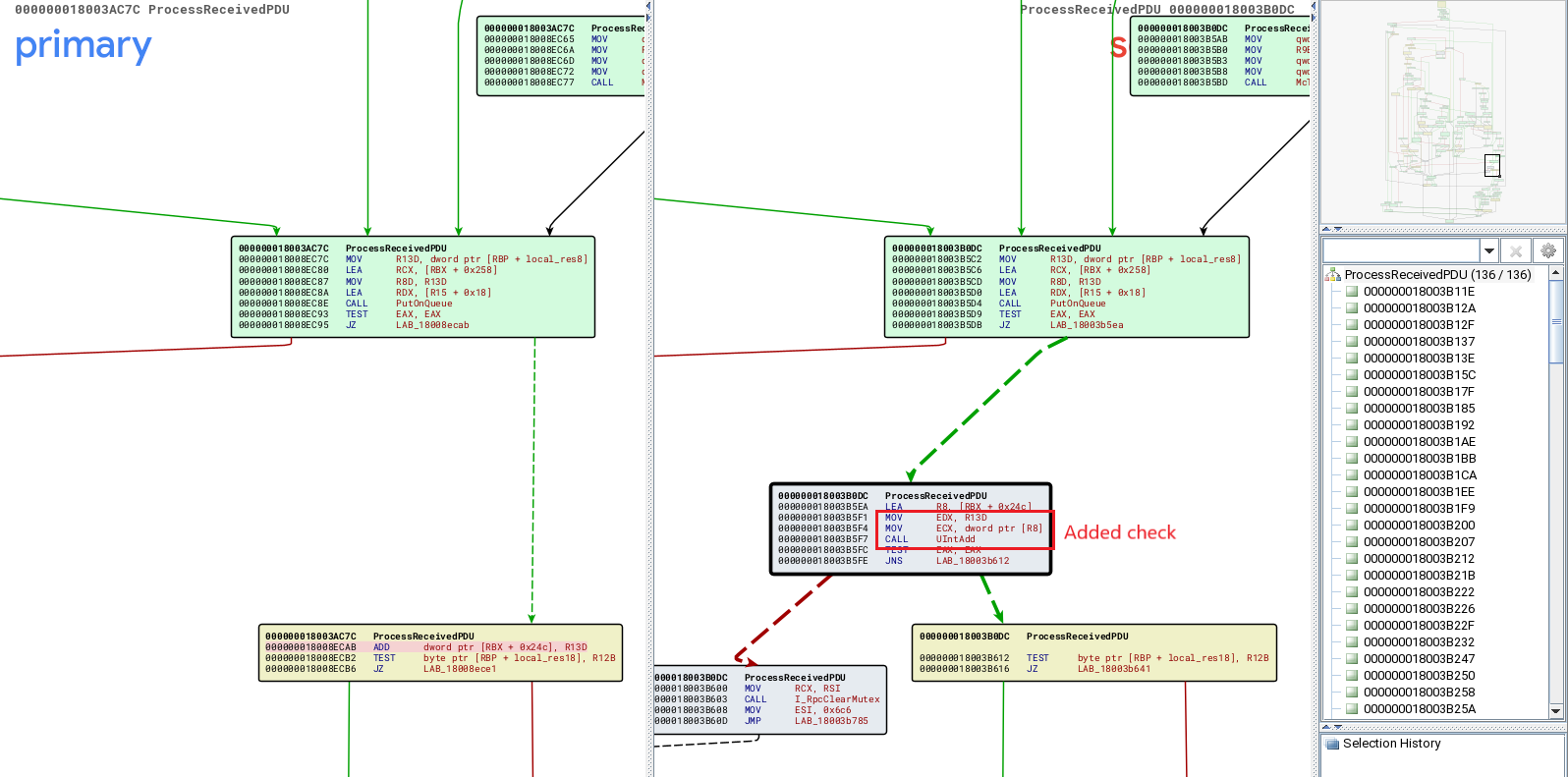So, seems that an integer overflow was the problem in the library.
Now, it’s required to find the answer to some questions:

1. Is the fix introduced in other functions?
2. How the bug could be reached, i.e. what we need to raise it?
3. How to gain command execution?

### The Patch

The fix has been introduced calling a function,i.e. UIntAdd(), to sum two items and check if integer overflow happened. The routine is very basic and it was present already in the vulnerable library but never called.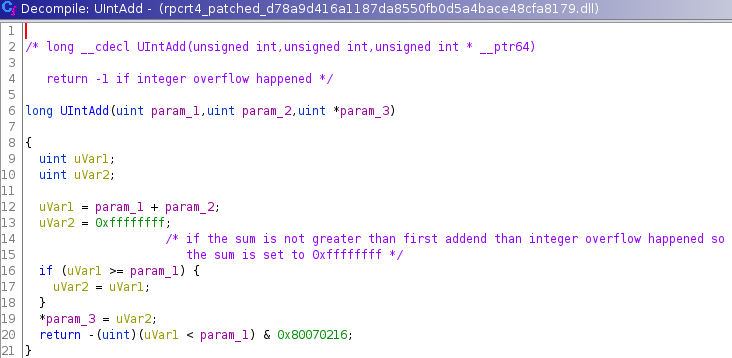The fix, as can be shown by call reference has been applied to multiple routines but in the vulnerable code the routine is never used.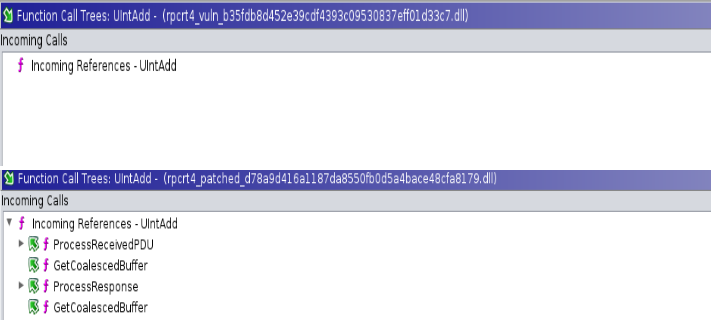At this point, due to multiple usage of `UIntAdd()` introduced I could assume that the bug was an integer overflow but I was not sure about which routine is effectively reachable in a easy way for a newbe like me.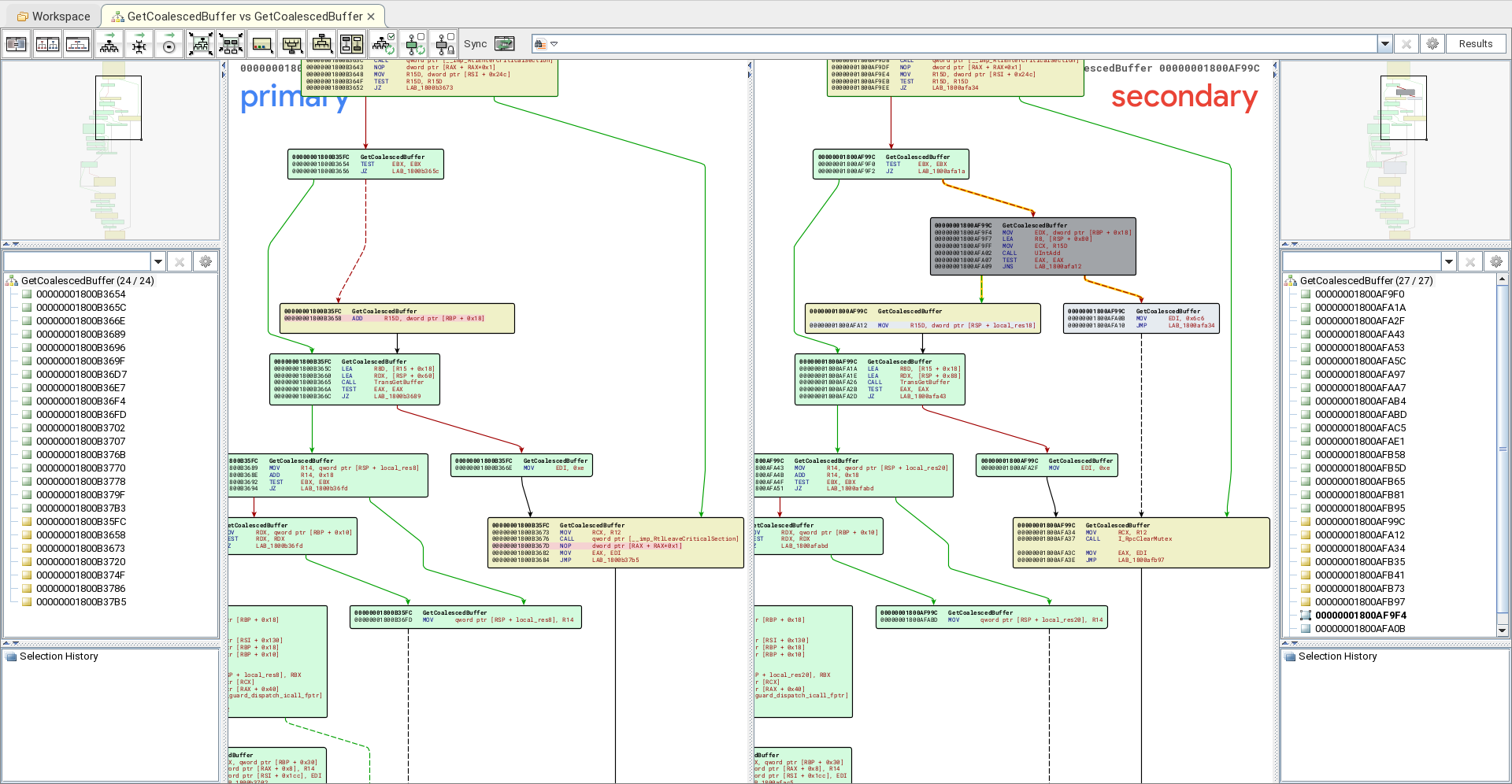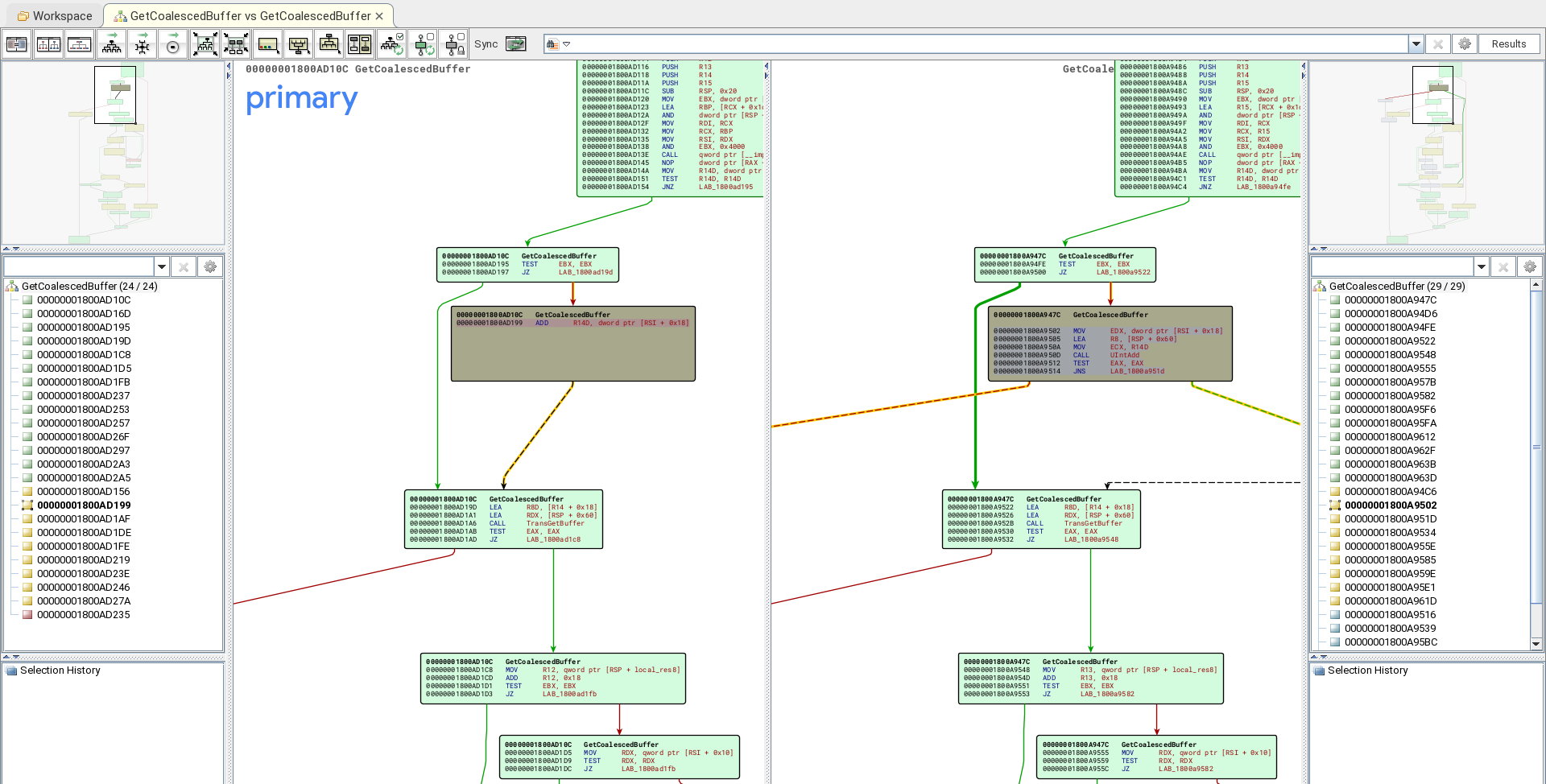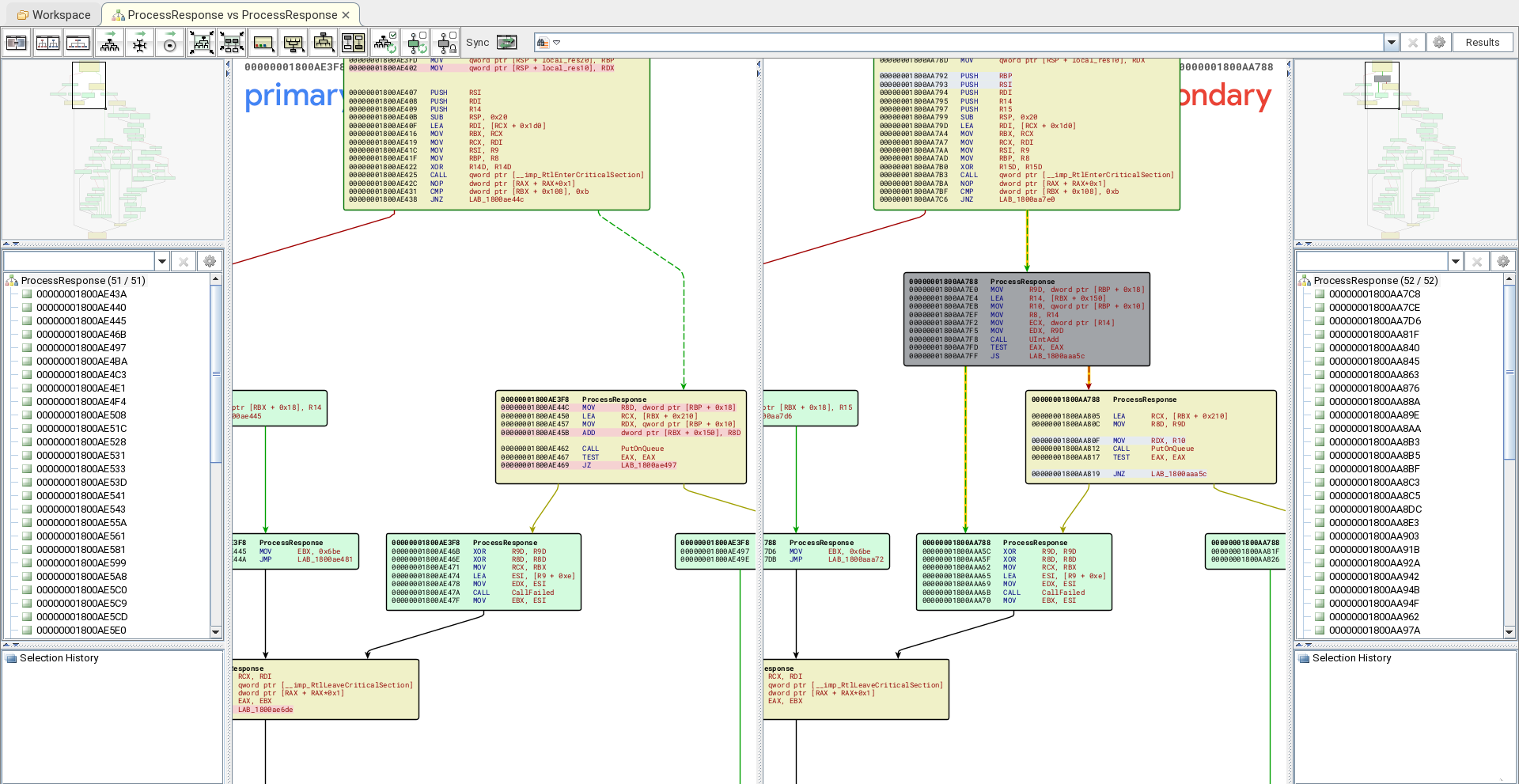## Search the way to reach the vulnerable point

Since my knowledge in RPC internals tending to 0 then for me the most easier approach was to build an RPC Client/Server example and sets some breakpoint on first instruction of the routines that contain the fix:

1. void OSF_CCONNECTION: OSF_CCALL::ProcessReceivedPDU(OSF_CCALL *this,void *param_1,int param_2) - offset: BaseLibrary + 0x3ac7c
2. long OSF_CCALL::GetCoalescedBuffer(OSF_CCALL *this,_RPC_MESSAGE *param_1) - offset BaseLibrary + 0xad10c
3. long OSF_CCALL::ProcessResponse(OSF_CCALL *this,rpcconn_response *param_1,_RPC_MESSAGE *param_2,int *param_3) - offset: BaseLibrary + 0xae3f8
4. long OSF_SCALL::GetCoalescedBuffer(OSF_SCALL *this,_RPC_MESSAGE *param_1,int param_2) - offset: BaseLibrary + 0xb35fc

The first example I run to check if the vulnerable code is reached in some way is a basic RPC client server with a Windows NT authentication and multiple kind of protocol selectable by arguments, the example used in this phase is visible at: Github

The github link above contains a basic server client RPC with customizable endpoint/protocol selection and basic authentication WINNT just on the connection, RPC_C_AUTHN_LEVEL_CONNECT.

The supported protocols are:

1. ncacn_np - named pipes are the medium Doc
2. ncacn_ip_tcp - TCP/IP is the stack on which the RPC messages are sent, indeed the endpoint is a server and port Doc
3. ncacn_http - IIS is the protocol family, the endpoint is specified with just a port number Doc
4. ncadg_ip_udp - UDP/IP is the protocol stack on which RPC messages are sent, this is obsolete.
5. ncalrpc - the protocol is the local interprocess communication, the endpoint is specified with a string at most 53 bytes long Doc

In my initial tests, I run the example, RPCServerTest.exe 1 5000 using the TCP/IP as protocol in order to inspect packets with wireshark. The RPC is based on some transport protocol that can be different and on top there is of course a common protocol used to call remote procedure, i.e. passing parameters serialized and choosing the routine to execute.

So, I run the example via x64dbg and put the breakpoints in the main functions that were patched, using the following script:

``````\$base_rpcrt4 = rpcrt4:base

\$addr = \$base_rpcrt4 + 0x3ac7c
log "Put BP on {addr} "

log "Put BP on {addr} "

\$addr = \$base_rpcrt4 + 0xae3f8
log "Put BP on {addr} "

\$addr = \$base_rpcrt4 + 0xb35fc
log "Put BP on {addr} "

``````

Running the client, RPCClientTest.exe 1 5000, the execution stops at OSF_SCALL::ProcessReceivedPDU().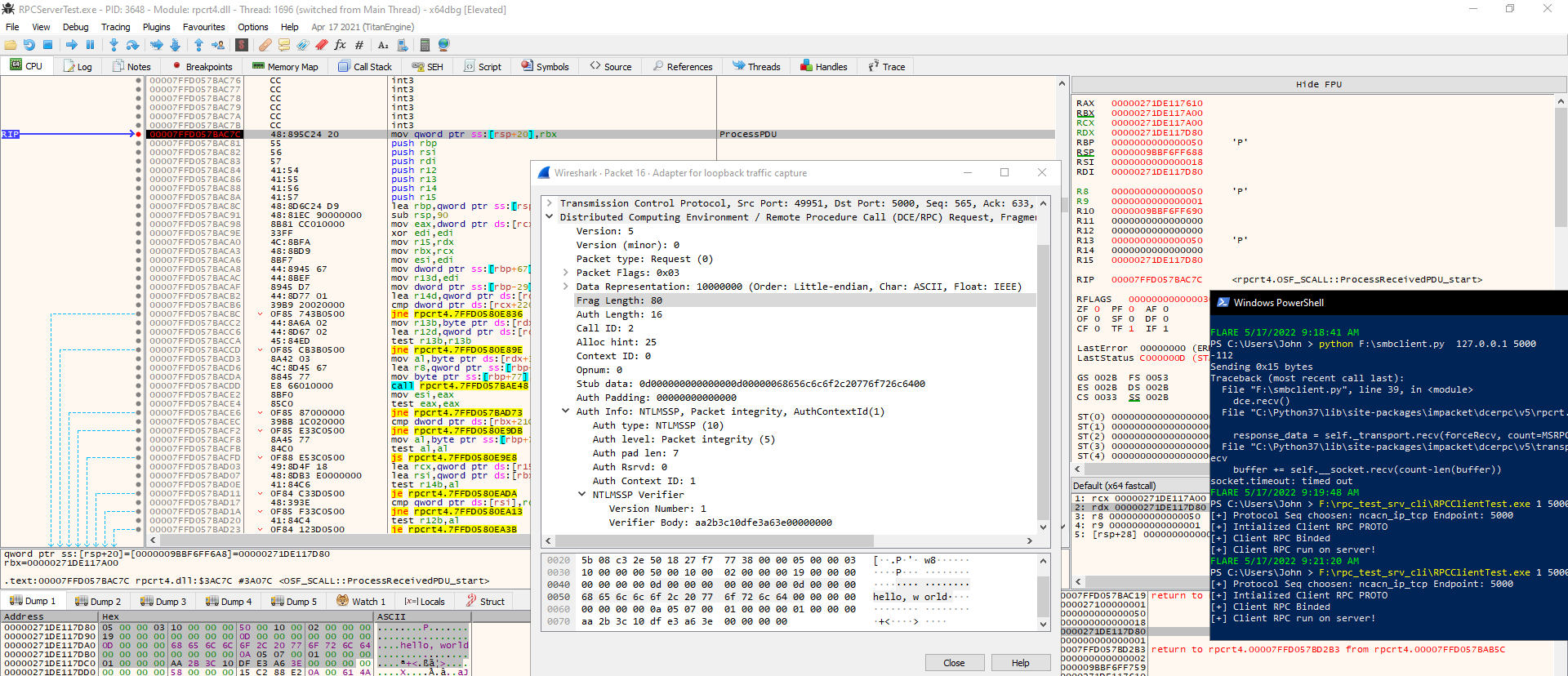The only vulnerable function touched with the previous test is: OSF_SCALL::ProcessReceivedPDU() and it is reached when the client ask for executing the hello procedure. Now it’s required to understand what is passed as argument to the function as arguments, just by the name it’s possible to assume that the routine is called to process the Protocol Data Unit received.

In order to understand how the function is called and so which are the parameters passed it’s required to analize the functions in the stack trace. Below is shown how in the previous test is reached the vulnerable routine.

``````OSF_SCALL::ProcessReceivedPDU(OSF_SCALL *param_1,OSF_SCALL **param_2,byte *param_3,int param_4)
rpcrt4.dll + 0x3ac14 #  OSF_SCALL::BeginRpcCall(longlong *param_1,OSF_SCALL **param_2,OSF_SCALL **param_3)
rpcrt4.dll + 0x3d2ae #  void OSF_SCONNECTION::ProcessReceiveComplete(longlong *param_1,longlong *param_2,OSF_SCALL **param_3,ulonglong param_4)
rpcrt4.dll + 4c99c   #  void DispatchIOHelper(LOADABLE_TRANSPORT *param_1,int param_2,uint param_3,void *param_4, uint param_5,OSF_SCALL **param_6,void *param_7)
other libraries
...
``````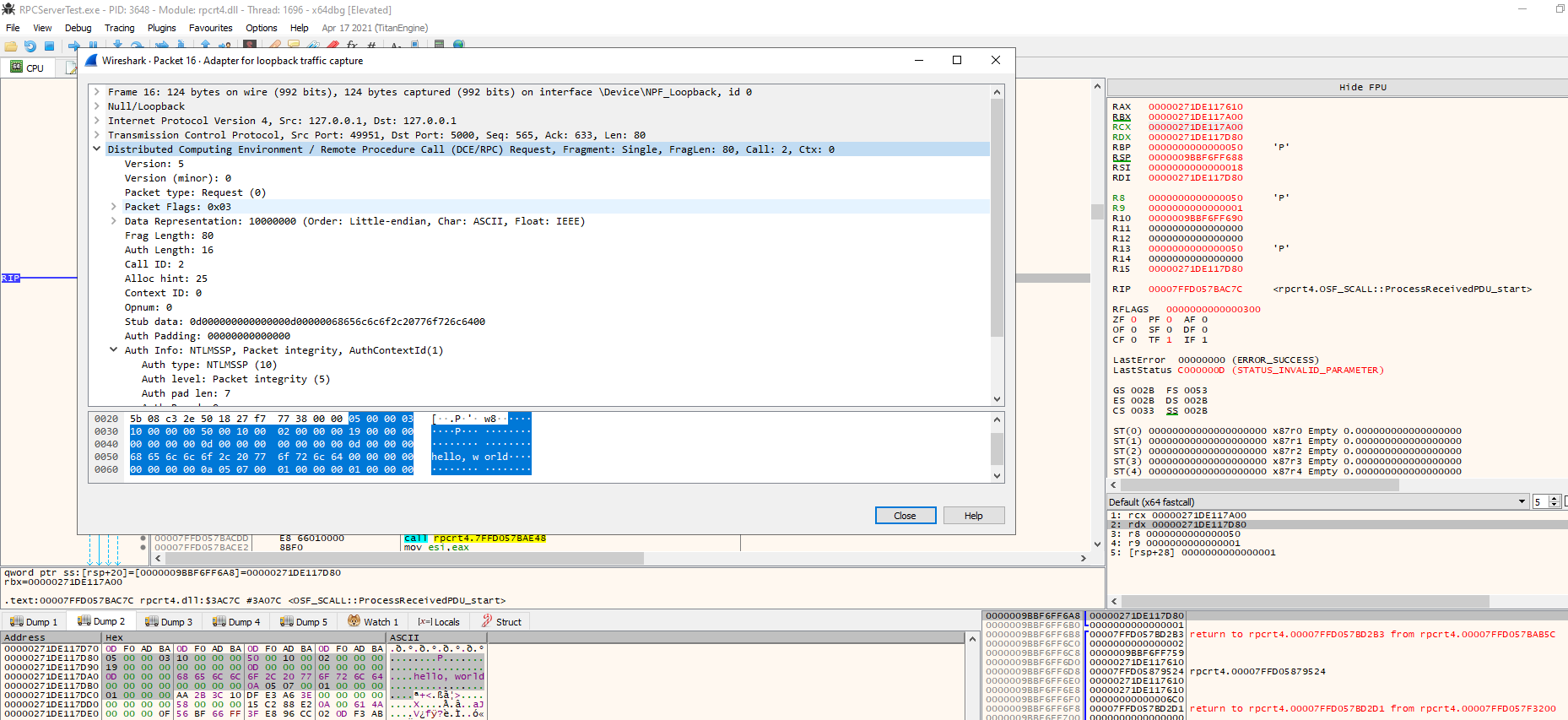The param1 of the `OSF_SCALL::ProcessReceivedPDU()` should be the class instance that maintain the status of the connection. The param2 of the `OSF_SCALL::ProcessReceivedPDU()` is just the tcp payload received from the client. The param3 of the `OSF_SCALL::ProcessReceivedPDU()` could be the tcp len field or the fragment len field, in any case seems that both are each time equals. The param4 of the `OSF_SCALL::ProcessReceivedPDU()` at first look should be something related the auth method choosen.

Unfortunately, seems that both param2 and param3 are passed directly to `DispatchIOHelper()` and as shown in the stack trace the `dispatchIOHelper()` is reached probably in some asynchronous way, maybe a thread wait for a messages from clients and start the dispatcher on every message received.

Before exploring the code I created a .h file containing the DCE RPC data structure in order to have a better view on ghidra.

`````` typedef struct
{
char version_major;
char version_minor;
char pkt_type;
char pkt_flags;
unsigned int data_repres;
unsigned short fragment_len;
unsigned short auth_len;
unsigned int call_id;
unsigned int alloc_hint;
unsigned short cxt_id;
unsigned short opnum;
char data; // it should be .fragment_len - 24 bytes long
} dce_rpc_t;
``````

Let’s explore the code to understand better what can be done and under which constraints.

The vulnerable code is the following: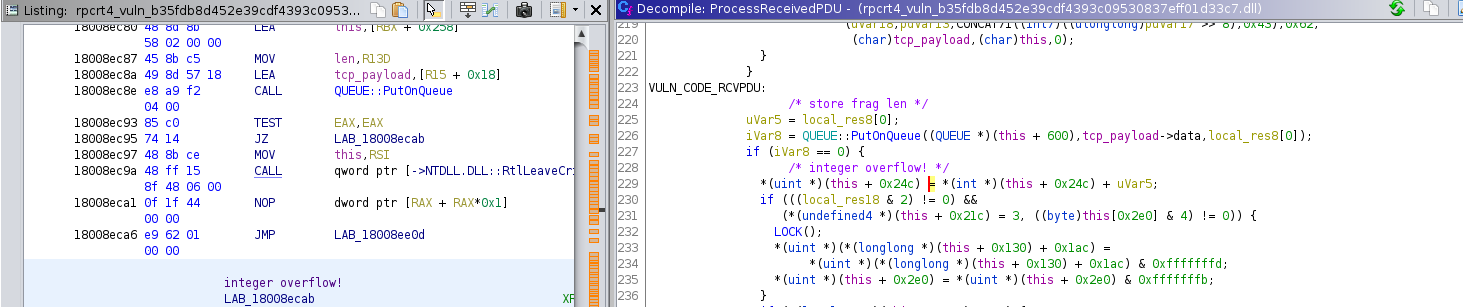In order to reach that part of the code is required to pass some check. It’s required to take this branch, this is taken every time. I don’t care why at the moment.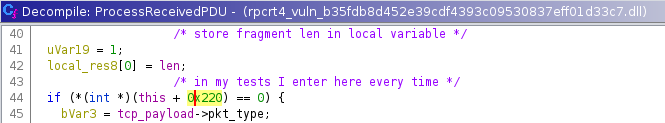The following branches should not be taken, so the packet sent should be a REQUEST packet in order to avoid those branches.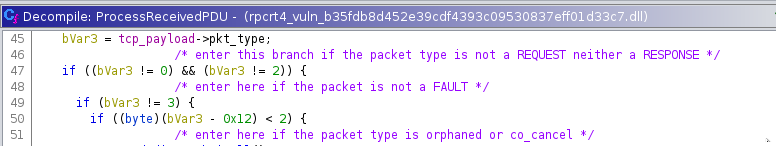It’s required to enter branch at 115 line, this is taken every time, maybe due to authentication.

The branch at line 117 in my tests is never taken.

The branch at line 119 should not be taken otherwise the data sent are overwritten, in order to not enter it’s required that the packet flags is not negative:

``````    8th bit the highest: Object <-- must be 0
7th bit            : Maybe
6th bit            : Did not execute
5th bit            : Multiplex
4th bit            : Reserved
3th bit            : Cancel
2th bit            : Last fragment
1th bit            : First fragment
``````

local_res18 is negative only if the highest bit is set, so in order to avoid that branch it’s required to not set Object flag.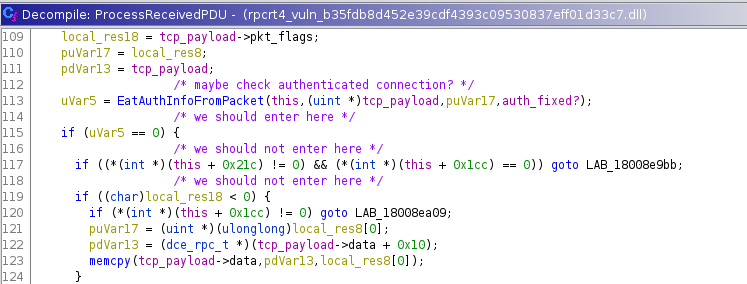It’s required to enter in the branch at line 128 so the packet sent should not be the first fragment and so it should not have the first fragment flag enabled. Since my tests until now have been conducted with this code Github, I decided to implement a client using Impacket library it is found here: Github.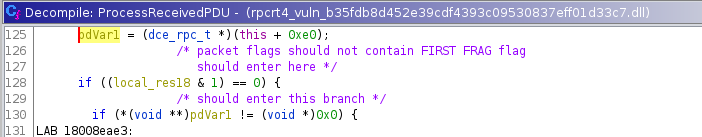Indeed with the initial test written in C, the al register, BP on base library + 0x3ad0e, containing the packet flags is set to 0x03, i.e. FIRST_FRAG & LAST_FRAG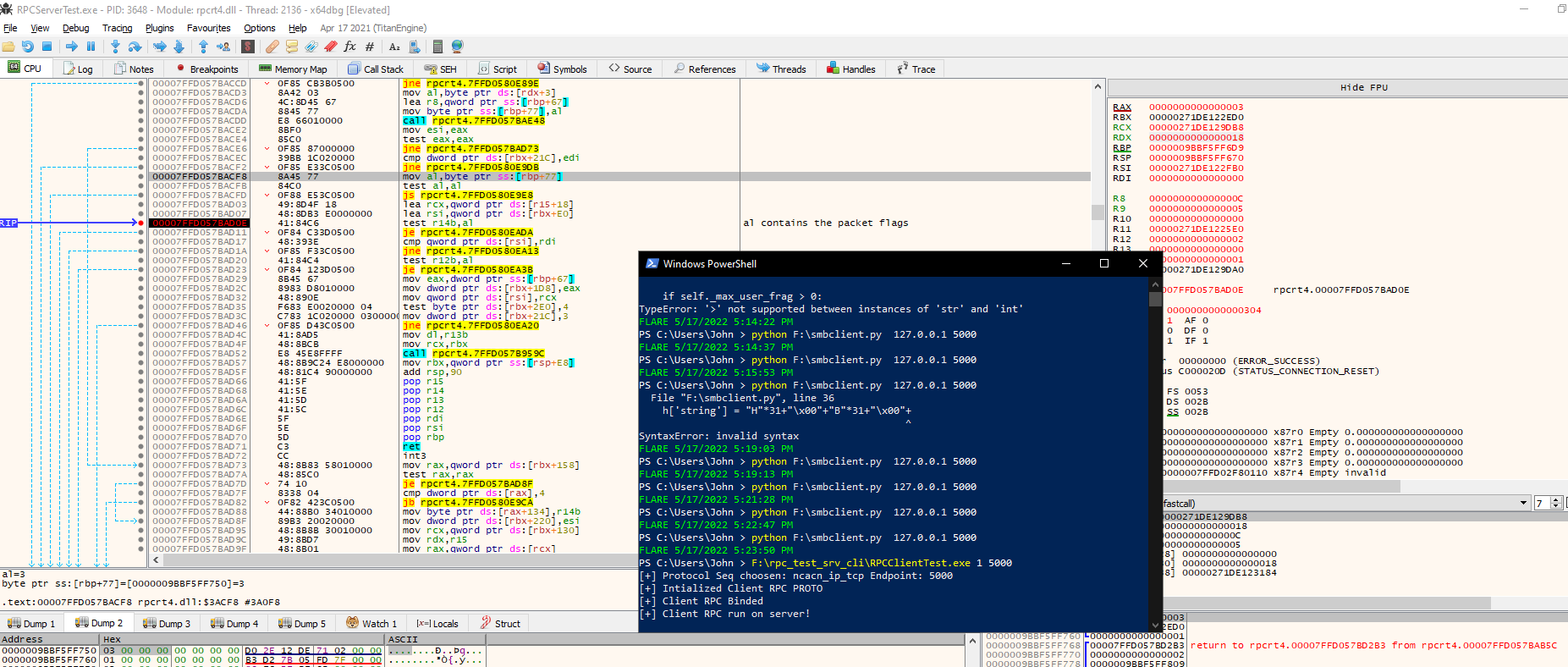On the second packet received with the impacket client al register contains 0 because the middle packet is not the first and neither the last of course.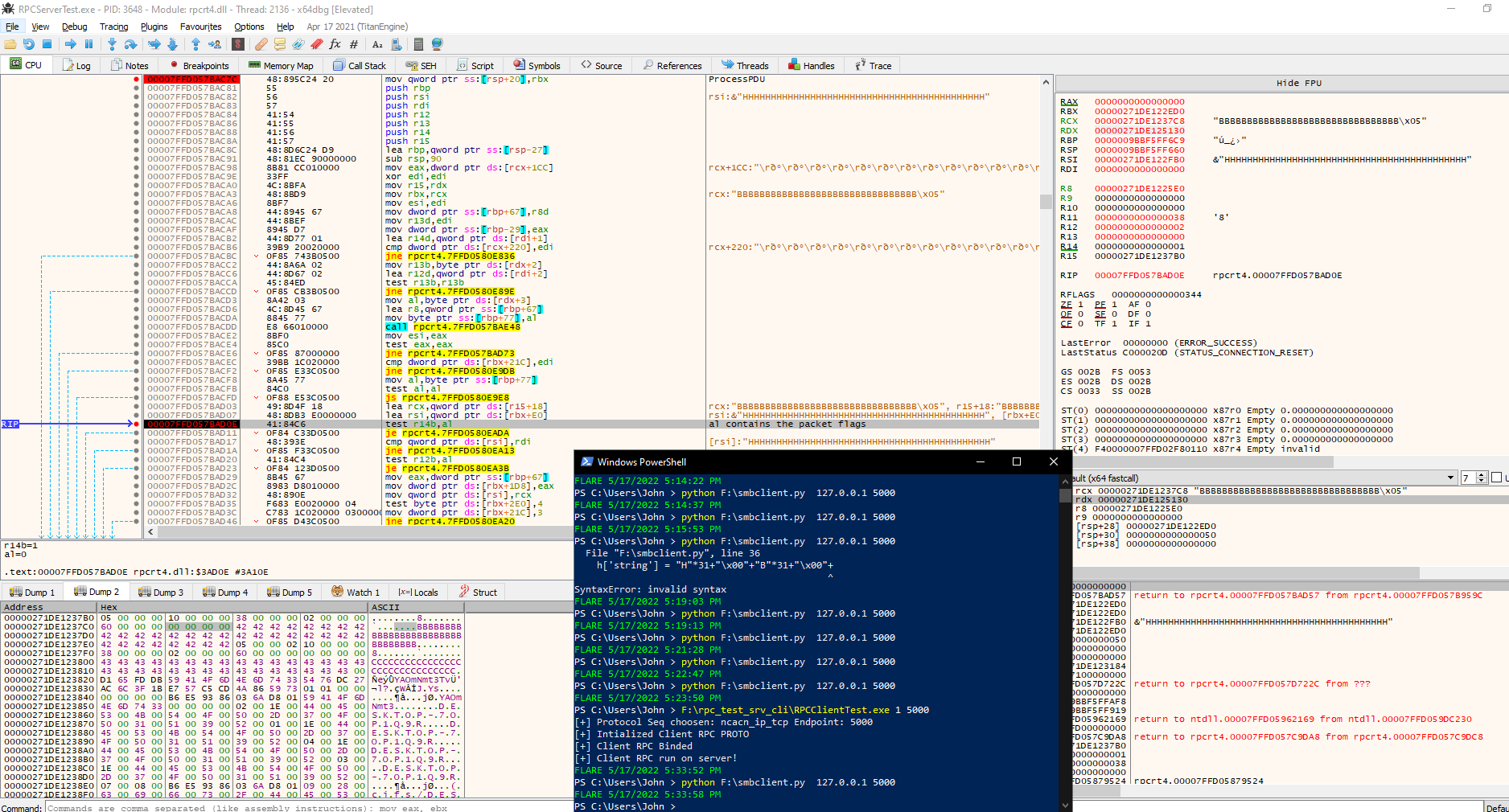The branch at line 130 is taken anytime, but the one at line 134 is never taken with any client. Not digged inside conditions that I did not need to bypass*

So, it’s required to force the following conditions :

``````if ((*(int *)(this + 0x244) == 0) || (*(int *)(this + 0x1cc) != 0))
``````

To avoid the branch `this+0x244` should be not 0 and `this + 0x1cc` should be 0. `this+0x244` is set to 1 in the function `void OSF_SCALL::BeginRpcCall(longlong *param_1,dce_rpc_t *tcp_payl,OSF_SCALL **param_3):baselibrary+0x8e806`.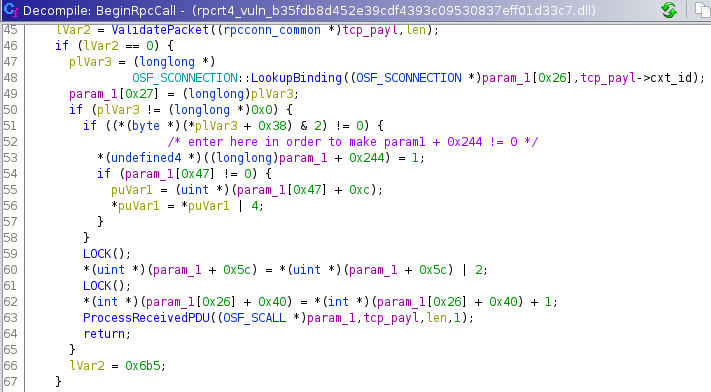I focused on the `*plVar3 + 0x38` value because `*this +0x244` is zeroed every time in `ActivateCall()`, called from `BeginRpcCall()`.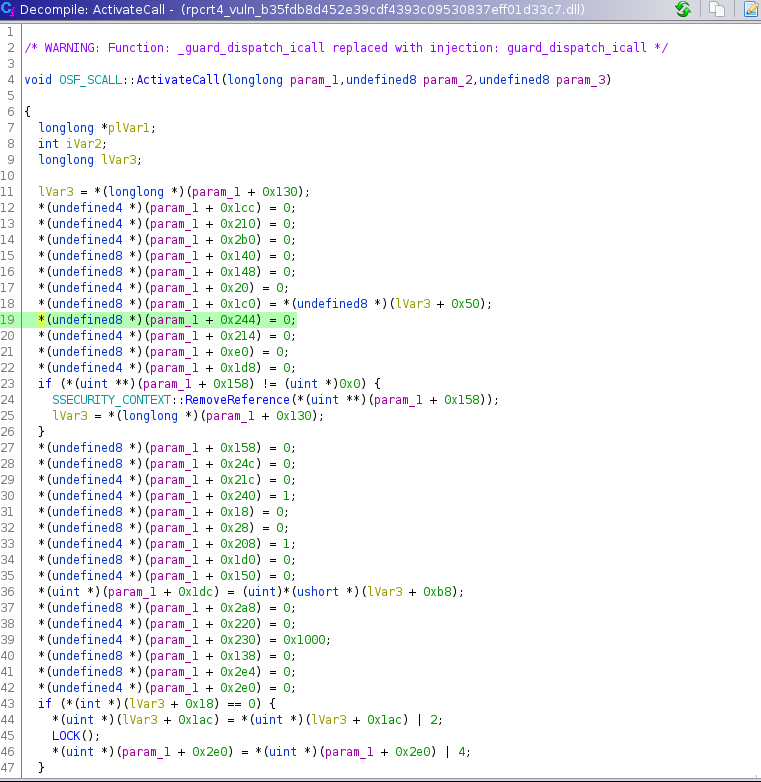`OSF_SCONNECTION::LookupBinding()` just walks a list until it finds the second parameter that corresponds to `context id` passed in the dce rpc packet.

``````OSF_SBINDING * __thiscall OSF_SCONNECTION::LookupBinding(OSF_SCONNECTION *this,ushort param_1)

{
OSF_SBINDING *pOVar1;
SIMPLE_DICT *this_00;
ulonglong uVar2;
uint local_res8 ;

local_res8 = 0;
this_00 = (SIMPLE_DICT *)(this + 0x80);
uVar2 = (ulonglong)param_1;
do {
pOVar1 = (OSF_SBINDING *)SIMPLE_DICT::Next(this_00,local_res8);
if (pOVar1 == (OSF_SBINDING *)0x0) {
return (OSF_SBINDING *)0x0;
}
} while (*(int *)(pOVar1 + 8) != (int)uVar2);
return pOVar1;
}
``````

So it gets every item from the list, stored at `OSF_CONNECTION instance + 0x80`.

``````void * __thiscall SIMPLE_DICT::Next(SIMPLE_DICT *this,uint *param_1)

{
void *pvVar1;
uint uVar2;
ulonglong uVar3;

uVar2 = *param_1;
if (uVar2 < *(uint *)(this + 8)) {
do {
uVar3 = (ulonglong)uVar2;
uVar2 = uVar2 + 1;
pvVar1 = *(void **)(*(longlong *)this + uVar3 * 8);
*param_1 = uVar2;
if (pvVar1 != (void *)0x0) {
return pvVar1;
}
} while (uVar2 < *(uint *)(this + 8));
}
*param_1 = 0;
return (void *)0x0;
}
``````

The routine that walks the dictionary shows the dictionary implementation, basically it contains the item number at dictionary+0x8 and every item is long just 8 bytes,indeed it is a memory address. The second parameter passed to the `Next() routine` is just an memory address pointing to a value used to check if the right item is found during the walk. So at the end the vulnerable code is reached if and only if the address returned from the lookup, i.e. `*plVar3 + 0x38` has second bit set

``````BeginRpcCall()
....
plVar3 = (longlong *)
OSF_SCONNECTION::LookupBinding((OSF_SCONNECTION *)param_1[0x26],tcp_payl->cxt_id); // get the item
param_1[0x27] = (longlong)plVar3;
if (plVar3 != (longlong *)0x0) {
if ((*(byte *)(*plVar3 + 0x38) & 2) != 0) {
/* enter here in order to make param1 + 0x244 != 0 */
*(undefined4 *)((longlong)param_1 + 0x244) = 1;
....
``````

The structures links starting from the connection instance structure to the structure containing the flag checked that could lead us to set `instance(OSF_SCONNECTION) + 0x244 to 1` is shown below.

``````typedef struct
{
...
offset 0x130: dictionary_t *dictionary;
...
offset 0x244: uint32_t flag; // must be 1 to enter in vulnerable code block in ProcessReceivedPDU()
...
} OSF_SCONNECTION_t

typedef struct
{
...
offset 0x80: items_list_t *itemslist;
...
}dictionary_t;

typedef struct
{
offset 0x0: item_t * items[items_no]
offset 0x8: uint32_t items_no;
...
}items_list_t

typedef struct
{
offset 0x0: flags_check_t *flags;
...
}item_t

typedef struct
{
...
offset 0x38: uint32_t flag_checked
...
}
``````

At this point it’s important to understand where it creates the dictionary value and where the value is set. I found that the dictionary address changes every connect. So it should be created on the client connection.

To find where the dictionary is assigned to `this[0x26]`, i.e. in the OSF_SCONNECTION instance, I placed a breakpoint on write on the memory address used to store the dictionary address, i.e. `this[0x26]`.

This unveils where the dictionary is inserted into the instance object.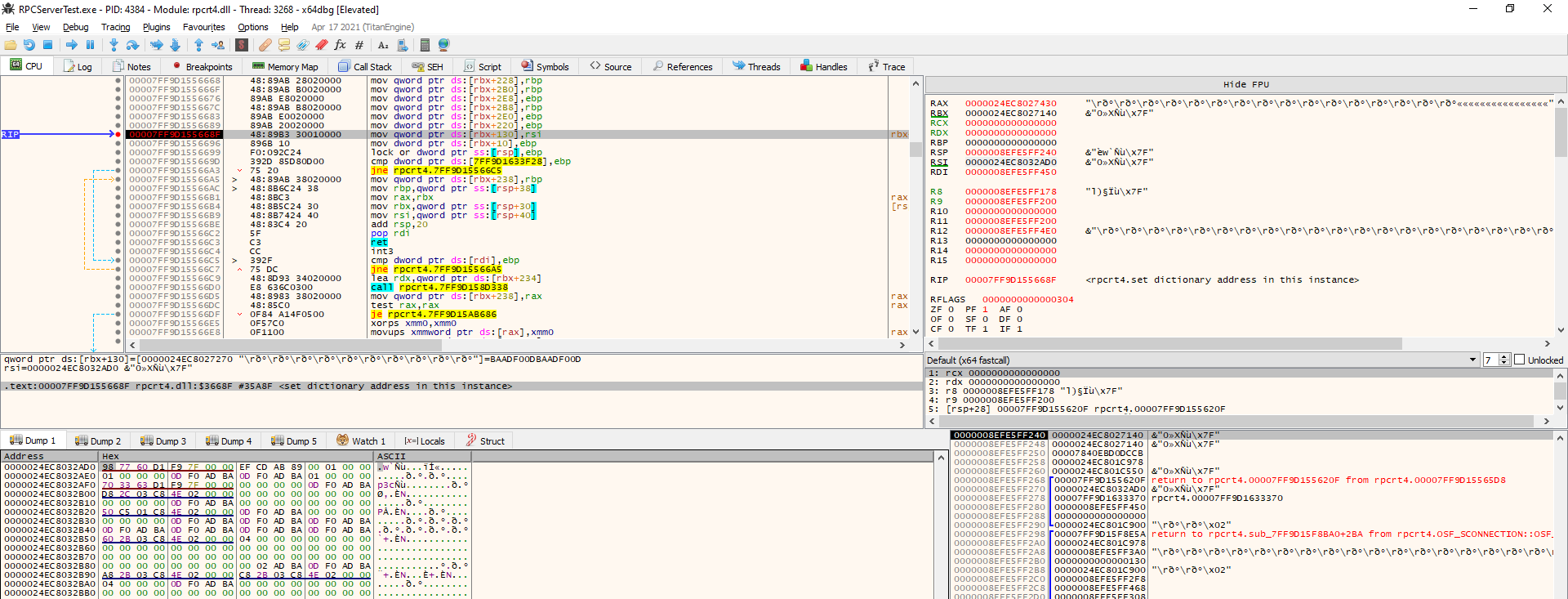Seems that it is created at: `rpcrt4.dll+0x3668f:OSF_SCALL::OSF_SCALL()`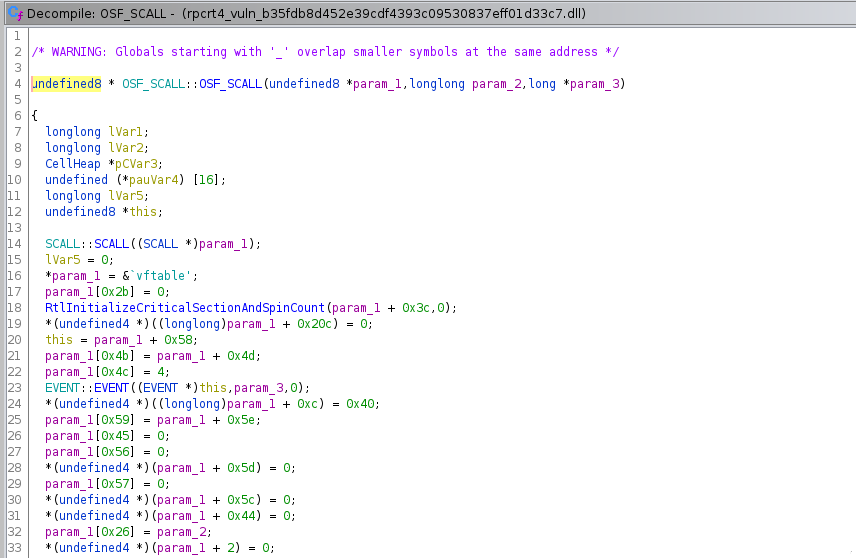Below the stack trace:

``````rpcrt4.dll+0x3668f:OSF_SCALL::OSF_SCALL()
rpcrt4.dll+0x3620a:OSF_SCONNECTION::OSF_SCONNECTION()
``````

Since, `LookupBinding(dictionary, item)`, searches the item starting from the `param1+0x80` and since there is just one client that is connecting.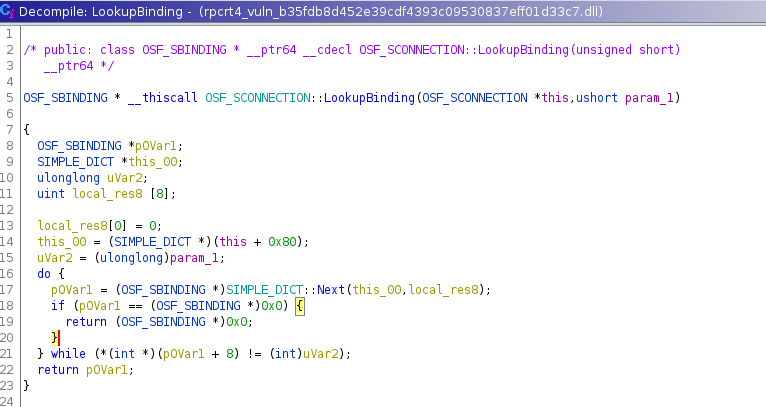Below is shown in the debugger the link that starts from the connection instance to the flag contained in the item returned from lookup.The dictionary is filled with an item already instantiated, i.e. with flag already set, in the `OSF_SCONNECTION::ProcessPContextList()` routine. Basically, this routine is reached from with the following stack:

``````rpcrt4.dll + 0x3e4f5: SIMPLE_DICT::Insert()
rpcrt4.dll + 0x39473: OSF_SCONNECTION::ProcessPContextList()
rpcrt4.dll + 0x38b31: OSF_SCONNECTION::AssociationRequested()
rpcrt4.dll + 0x3d3b6: OSF_SCONNECTION::processReceiveComplete()
``````

`OSF_SCONNECTION::processReceiveComplete()` is called every time arrive a packet from the client. The S in front of the namespace means that the routine is used by the RPC servers.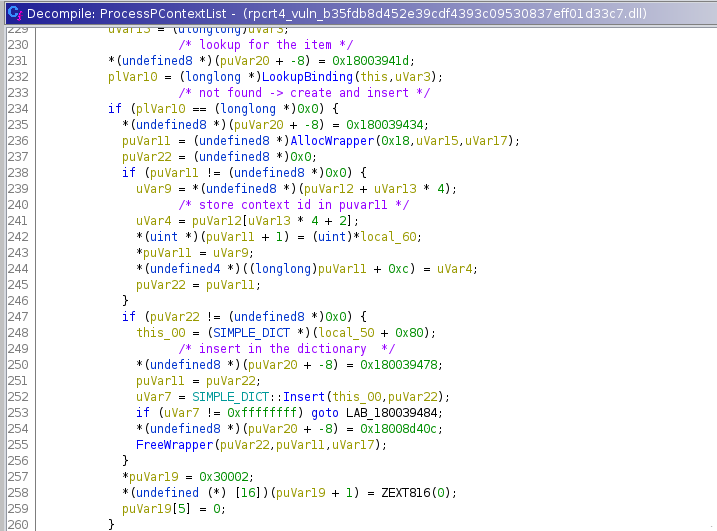This routine, `OSF_SCONNECTION::ProcessPContextList()`, store the `context id` in a new dictionary item, from this routine could be found the exact structure of the dictionary items at `instance(OSF_SCONNECTION)[0x26]`. Unfortunately the item, i.e. the structure that contains the flag to force is not initialized on every connection.

Indeed I added a break point on the flag memory address to track the instructions overwriting it. I would expect some free/alloc on every connection just like the dictionary, but this never happened. This led me to think that it should be created during RPC Server initialization.

The item address, i.e. the object that contains the flag to force, is retrieved and set in the dictionary at:

``````0x57b54 RPC_SERVER::FindInterfaceTransfer()

offset: 0x57a6c | mov rsi,qword ptr ds:[GlobalRpcServer]          ; RSI will contain the address of GlobalRPCServer
offset: 0x57aae | mov rdx,qword ptr ds:[rsi+120]                  ; most likely address of items
offset: 0x57ab9 | mov rdi,qword ptr ds:[rdx+rax*8]                ; get the ith item
offset: 0x57b30 | call RPC_INTERFACE::SelectTransferSyntax        ; check if the connection match with the interface
offset: 0x57b35 | test eax,eax                                    ; if eax == 0 return, interface/item found!
offset: 0x57b54 | mov qword ptr ds:[rax],rdi                      ; Address of the item with the flag to force is returned writing it in *rax
``````

Basically, `RPC_SERVER::FindInterfaceTransfer()` is called by `ProcessPContextList()` and it is executed most likely to find the RPC interface according to the information sent by the client for example, uuid value.

Below the debugger view on `0x57b54`.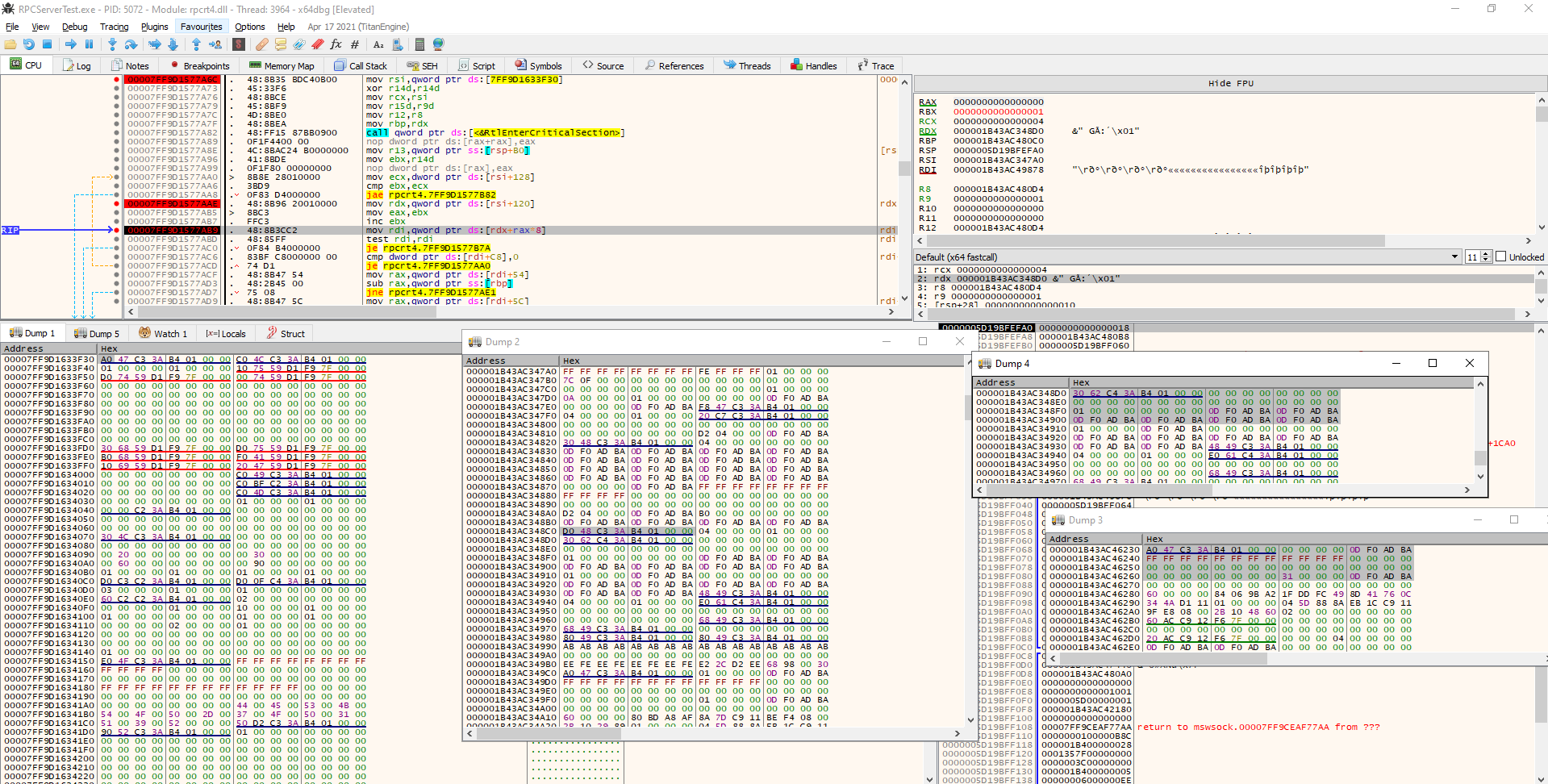Since `GlobalRpcServer` is fixed address, and it contains the address of the struct that contain the items, I just looked at the write reference on ghidra and found where `GlobalRpcServer` is filled with the struct address.

``````wchar_t ** InitializeRpcServer(undefined8 param_1,uchar **param_2,SIZE_T param_3)
{
ppwVar24 = (wchar_t **)0x0;
local_res8._0_4_ = 0;
ppwVar19 = ppwVar24;
if (GlobalRpcServer == (RPC_SERVER *)0x0) {
this = (RPC_SERVER *)AllocWrapper(0x1f0,param_2,param_3);
ppwVar5 = ppwVar24;
if (this != (RPC_SERVER *)0x0) {
param_2 = local_res8;
ppwVar5 = (wchar_t **)RPC_SERVER::RPC_SERVER(this,(long *)param_2);
ppwVar19 = (wchar_t **)(ulonglong)(uint)local_res8;
}
if (ppwVar5 == (wchar_t **)0x0) {
GlobalRpcServer = (RPC_SERVER *)ppwVar5;
return (wchar_t **)0xe;
}
GlobalRpcServer = (RPC_SERVER *)ppwVar5; // OFFSET: 0xb3d2; here the global rpc server is set to a new fresh memory.
...
}
...
}
``````

The routine `InitializeRpcServer()` is called from a `RpcServerUseProtseqEpW()` that is directly called by the RPC server! As shown in the debugger view, `*GlobalRpcServer + 0x120` is already filled but `*(*GlobalRpcServer + 0x120)` is equal to NULL.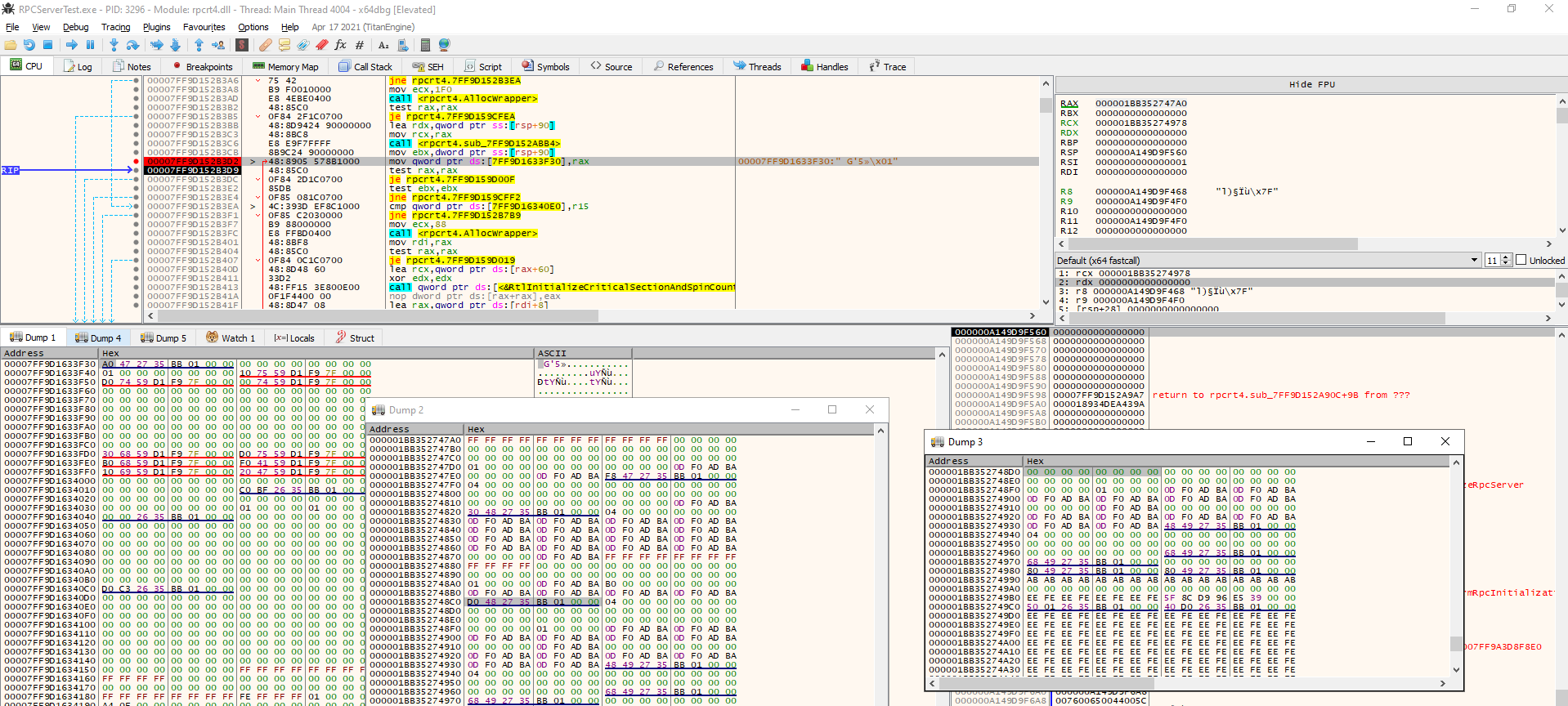Setting a breakpoint on memory write of: `*(*GlobalRpcServer + 0x120)` , I found where the address of the item is set! Below is shown the stack trace:

``````rpcrt4.dll + 0x3e4f9 at SIMPLE_DICT::Insert()
rpcrt4.dll + 0xd498  at RPC_INTERFACE * RPC_SERVER::FindOrCreateInterfaceInternal()
rpcrt4.dll + 0xd196  at void RPC_SERVER::RegisterInterface()
rpcrt4.dll + 0x7271e at void RpcServerRegisterIf()
server rpc function
``````

From the memory contents shown below: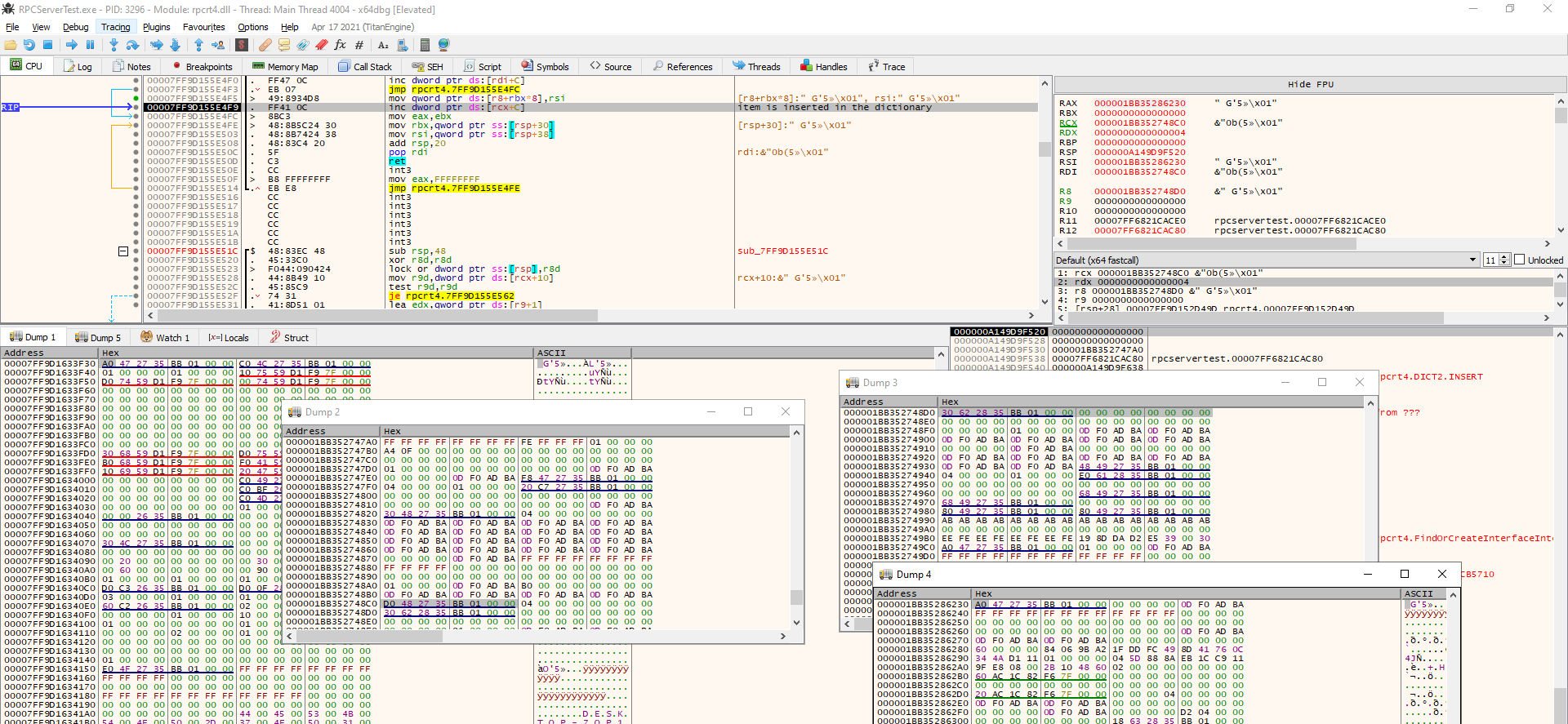The `*item + 0x38` appears to be NULL, let’s set a breakpoint to that memory address. To resume up, the flag to force is reachable from:

``````typedef struct
{
}GlobalRpcServer_t;

typedef struct
{
...
offset 0x120:  item_t **items
...
}rpc_interfaces_t;

typedef struct
{
...
offset 0x38:  uint32_t flag_checked // Flag check in BeginRpcCall()
...
}item_t
``````

It was clear that our flag to force has not already set so I set up another write breakpoint on `*item + 0x38` to find where it is set! So, I found the point where the flag is modified, as visibile in the debugger.The code that modifies the flag is reached from this stack trace.

``````rpcrt4.dll + 0xd30e : RPC_INTERFACE::RegisterTypeManager()
rpcrt4.dll + 0xd1bf : void RPC_SERVER::RegisterInterface()
rpcrt4.dll + 0x7271e: void RpcServerRegisterIf()
RPC server functions

void RpcServerRegisterIf(uint *param_1,uint *uuid,SIZE_T param_3)

{
...
RPC_SERVER::RegisterInterface
(GlobalRpcServer,param_1,uuid,param_3,0,0x4d2,gMaxRpcSize,(FuncDef2 *)0x0,
(ushort **)0x0,(RPCP_INTERFACE_GROUP *)0x0);

...
}

void RPC_SERVER::RegisterInterface
(RPC_SERVER *param_1,uint *param_2,uint *param_3,SIZE_T param_4,uint param_5,
uint param_6,uint param_7,FuncDef2 *param_8,ushort **param_9,
RPCP_INTERFACE_GROUP *param_10)

{
if (param_3 != (uint *)0x0) {
local_80 = param_3;
}
local_58 = ZEXT816(0);
local_78 = param_2;
local_60 = param_3;
...
puVar6 = RPC_INTERFACE::RegisterTypeManager(pRVar5,local_60,param_4);
...
}

RPC_INTERFACE::RegisterTypeManager(RPC_INTERFACE *param_1,undefined4 *param_2,SIZE_T param_3)
{
...
if ((param_2 == (undefined4 *)0x0) ||
(iVar6 = RPC_UUID::IsNullUuid((RPC_UUID *)param_2), iVar6 != 0)) {
if ((*(uint *)(param_1 + 0x38) & 1) == 0) {
*(int *)(param_1 + 200) = *(int *)(param_1 + 200) + 1;
*(uint *)(param_1 + 0x38) = *(uint *)(param_1 + 0x38) | 1; // SET to 1 the flag!
*(SIZE_T *)(param_1 + 0x40) = param_3;
RtlLeaveCriticalSection(pRVar1);
return (undefined4 *)0x0;
}
puVar11 = (undefined4 *)0x6b0;
}
...
}
``````

Looking at the stack trace and the code, it’s clear that the RPC server implementation called with `RpcServerRegisterIf()` without specifying any `MgrTypeUuid`. At this point it was most likely that I could, from client side, alter in some way that flag that trigger the vulnerable code.

Anyway, backing to the BeginRpcCall(), in order to execute vulnerable code it’s required that the item_t flag value has the second bit set. Since that value is never written, I could not use the debugger anymore to find where the second bit’s flag is set. So, I searched for instruction patterns basing on the information I got:

1. The value is bitmask flag, this was clear because the values are checked with TEST instruction and because the value, i.e. 1 has been set with an or operator.
2. The `item_t` is a kind of structure, most likely the access to the flag member more or less the same, i.e. `[register + 0x38]`
3. Flag is 4 byte long

According to these information I searched for instruction like: `or dword ptr [anyregs+0x38], 2`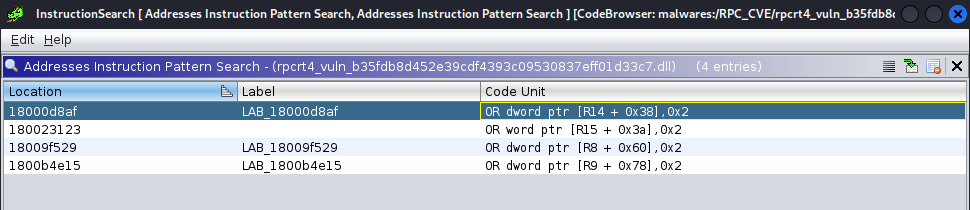``````RPC_INTERFACE::RPC_INTERFACE
(RPC_INTERFACE *this,_RPC_SERVER_INTERFACE *param_1,RPC_SERVER *param_2,uint param_3,
uint param_4,uint param_5,FuncDef2 *param_6,void *param_7,long *param_8,
RPCP_INTERFACE_GROUP *param_9)

{
RPC_INTERFACE *pRVar1;
int iVar2;
int iVar3;

*(undefined4 *)(this + 8) = 0;
RtlInitializeCriticalSectionAndSpinCount(this + 0x10,0);
*(undefined4 *)(this + 0x38) = 0;
*(undefined4 *)(this + 200) = 0;
pRVar1 = this + 0x170;
*(undefined (**) )(this + 0xd8) = (undefined (*) )(this + 0xe8);
*(undefined8 *)(this + 0xe0) = 4;
*(undefined (*) )(this + 0xe8) = ZEXT816(0);
*(undefined (*) )(this + 0xf8) = ZEXT816(0);
*(undefined4 *)(this + 0x14c) = 0;
*(undefined4 *)(this + 0x150) = 0;
*(undefined4 *)(this + 0x154) = 0;
*(undefined4 *)(this + 0x158) = 0;
*(undefined4 *)(this + 0x15c) = 0;
*(undefined4 *)(this + 0x160) = 0;
*(undefined4 *)(this + 0x164) = 0;
*(undefined4 *)(this + 0x168) = 0;
*(undefined4 *)(this + 0x16c) = 0;
RtlInitializeSRWLock(this + 0x1e0);
*(RPC_INTERFACE **)(this + 0x178) = pRVar1;
*(RPC_INTERFACE **)pRVar1 = pRVar1;
*(undefined4 *)(this + 0x180) = 0;
*(undefined4 *)(this + 0x1e8) = 0;
*(undefined (**) )(this + 0x1f0) = (undefined (*) )(this + 0x200);
*(undefined8 *)(this + 0x1f8) = 4;
*(undefined (*) )(this + 0x200) = ZEXT816(0);
*(undefined (*) )(this + 0x210) = ZEXT816(0);
*(undefined4 *)(this + 0x220) = 0;
*(undefined8 *)(this + 0x228) = 0;
*(RPC_SERVER **)this = param_2;
*(undefined8 *)(this + 0xd0) = 0;
*(undefined8 *)(this + 0xb8) = 0;
*(undefined8 *)(this + 0x230) = 0;
*(undefined8 *)(this + 0x238) = 0;
iVar3 = UpdateRpcInterfaceInformation
((longlong)this,(uint *)param_1,(ushort **)(ulonglong)param_3,param_4,param_5,
(longlong)param_6,(ushort **)param_7,(longlong)param_9);
iVar2 = *(int *)param_1;
*param_8 = iVar3;
if ((iVar2 == 0x60) && (((byte)param_1[0x58] & 1) != 0)) {
*(uint *)(this + 0x38) = *(uint *)(this + 0x38) | 2;
}
return this;
}
``````

Seems that the or is executed under some conditions. At this point, it was required to verify this assumption, I set up a breakpoint on those conditions: `offset: 0xd87c`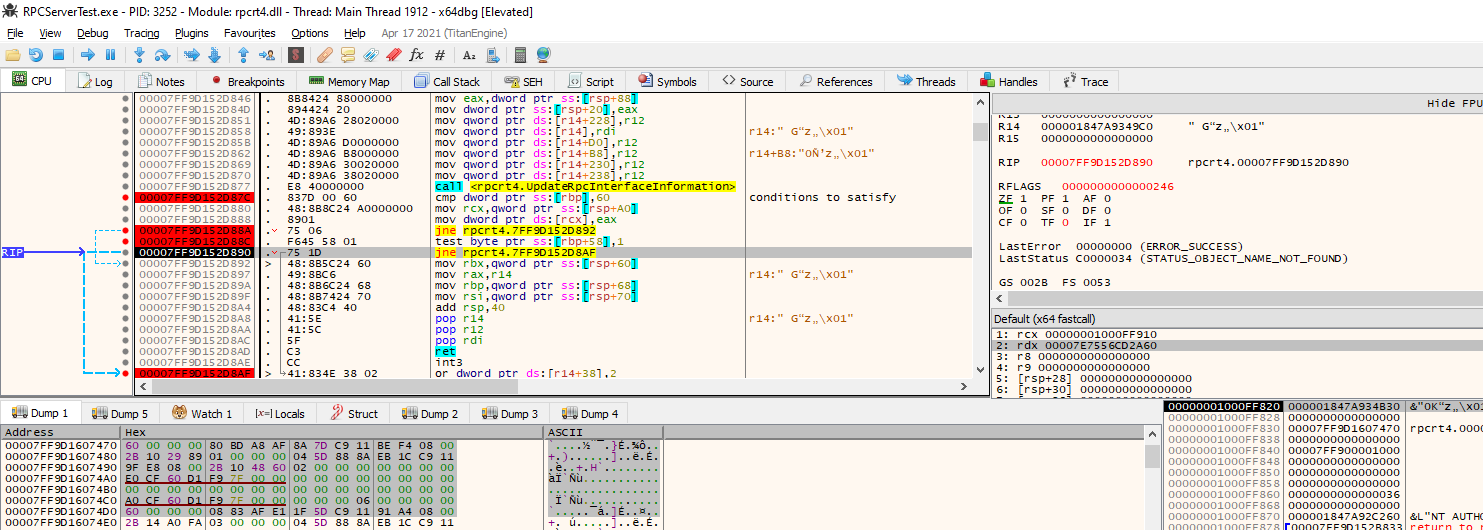``````rpcrt4.dll + 0xd87c at RPC_INTERFACE::RPC_INTERFACE()
rpcrt4.dll + 0xb82e at InitializeRpcServer()
rpcrt4.dll + x      at PerformRpcInitialization()
rpcrt4.dll + y      at RpcServerUseProtseqEpW()

wchar_t ** InitializeRpcServer(undefined8 param_1,uchar **param_2,SIZE_T param_3)
{
...
this_01 = (RPC_INTERFACE *)
RPC_INTERFACE::RPC_INTERFACE
(this_00,(_RPC_SERVER_INTERFACE *)&DAT_1800e7470,GlobalRpcServer,1,0x4d2,
0x1000,(FuncDef2 *)0x0,(void *)0x0,(long *)local_res8,
(RPCP_INTERFACE_GROUP *)0x0);
...
}
``````

The parameter on which the condition is check is fixed, i.e. `DAT_1800e7470`, and never altered.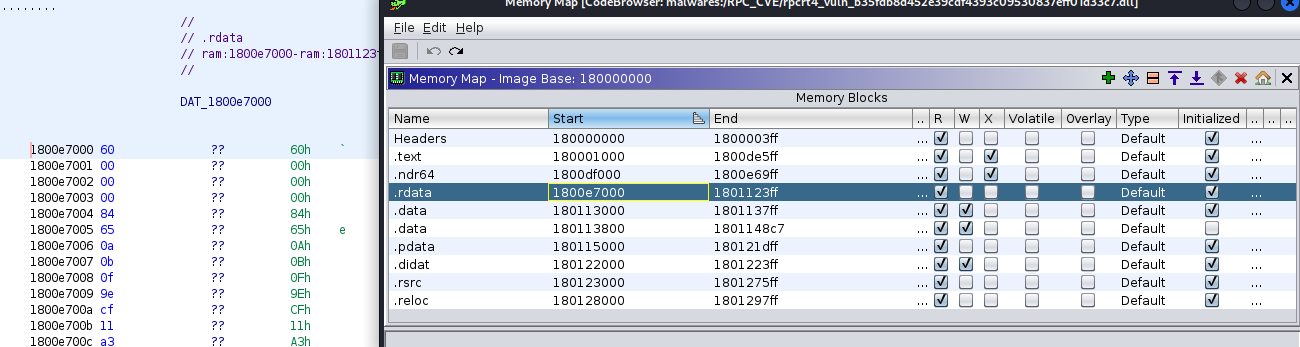But, going on with the debugger, I break on the same point. This time with another stack trace that starts exactly in my rpc server test!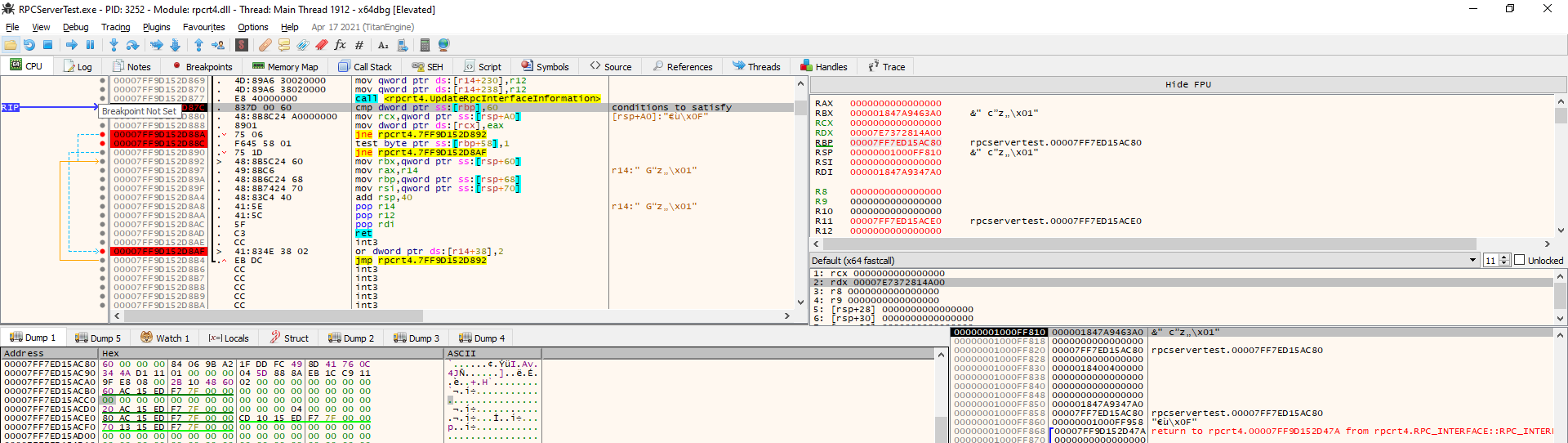``````rpcrt4.dll + 0xd87c  at RPC_INTERFACE::RPC_INTERFACE()
rpcrt4.dll + 0xd475  at RPC_INTERFACE * RPC_SERVER::FindOrCreateInterfaceInternal()
rpcrt4.dll + 0xd196  at void RPC_SERVER::RegisterInterface()
rpcrt4.dll + 0x7271e at void RpcServerRegisterIf()
RPCServerTest.exe: main

RPC_INTERFACE *
RPC_SERVER::FindOrCreateInterfaceInternal
(RPC_SERVER *param_1,_RPC_SERVER_INTERFACE *param_2,ulonglong param_3,uint param_4,
uint param_5,FuncDef2 *param_6,void *param_7,long *param_8,int *param_9,
RPCP_INTERFACE_GROUP *param_10)

{
...
pRVar5 = (RPC_INTERFACE *)AllocWrapper(0x240,p_Var8,uVar10);
uVar2 = (uint)p_Var8;
this = pRVar9;
if (pRVar5 != (RPC_INTERFACE *)0x0) {
p_Var8 = param_2;
this = (RPC_INTERFACE *)
RPC_INTERFACE::RPC_INTERFACE
(pRVar5,param_2,param_1,(uint)param_3,param_4,param_5,param_6,param_7,param_8,
param_10);
uVar2 = (uint)p_Var8;
}
...
}

void RPC_SERVER::RegisterInterface
(RPC_SERVER *param_1,uint *param_2,uint *param_3,SIZE_T param_4,uint param_5,
uint param_6,uint param_7,FuncDef2 *param_8,ushort **param_9,
RPCP_INTERFACE_GROUP *param_10)

{
...
RtlEnterCriticalSection(param_1);
pRVar5 = FindOrCreateInterfaceInternal
(param_1,(_RPC_SERVER_INTERFACE *)param_2,(ulonglong)param_5,param_6,local_84
,param_8,param_9,(long *)&local_80,(int *)&local_78,local_68);
...
}

void RpcServerRegisterIf(uint *param_1,uint *uuid,SIZE_T param_3)
{
MUTEX *pMVar1;
void *in_R9;
undefined4 in_stack_ffffffffffffffc8;
undefined4 in_stack_ffffffffffffffcc;

/* 0x726c0  1483  RpcServerRegisterIf */
if ((RpcHasBeenInitialized != 0) ||
(pMVar1 = PerformRpcInitialization
((int)param_1,uuid,(int)param_3,in_R9,
(void *)CONCAT44(in_stack_ffffffffffffffcc,in_stack_ffffffffffffffc8)),
(int)pMVar1 == 0)) {
RPC_SERVER::RegisterInterface
(GlobalRpcServer,param_1,uuid,param_3,0,0x4d2,gMaxRpcSize,(FuncDef2 *)0x0,
(ushort **)0x0,(RPCP_INTERFACE_GROUP *)0x0);
}
return;
}
``````

Basically, that conditions failed because of the first parameter passed by my rpc server test application to `RpcServerRegisterIf()`.

At this point I looked at my code to find which kind of configuration I used and how I could change it in My test server app.

``````API prototype >
RPC_STATUS RpcServerRegisterIf(
RPC_IF_HANDLE IfSpec,
UUID          *MgrTypeUuid,
RPC_MGR_EPV   *MgrEpv
);

My RpcServerRegisterIf call >
status = RpcServerRegisterIf(interfaces_v1_0_s_ifspec,
NULL,
NULL);

Value of interfaces_v1_0_s_ifspec >
static const RPC_SERVER_INTERFACE interfaces___RpcServerInterface =
{
sizeof(RPC_SERVER_INTERFACE),
,{1,0}},
,{2,0}},
(RPC_DISPATCH_TABLE*)&interfaces_v1_0_DispatchTable,
0,
0,
0,
&interfaces_ServerInfo,
0x04000000 // param_1[0x58]
};
``````

Of course, the value of `interfaces_v1_0_s_ifspec` is equal to the one shown in the last debugger image! Since, the failing check is done on `param_1[0x58] & 1`, I changed `0x04000000 to 0x04000001` in order to have the vulnerable code executing!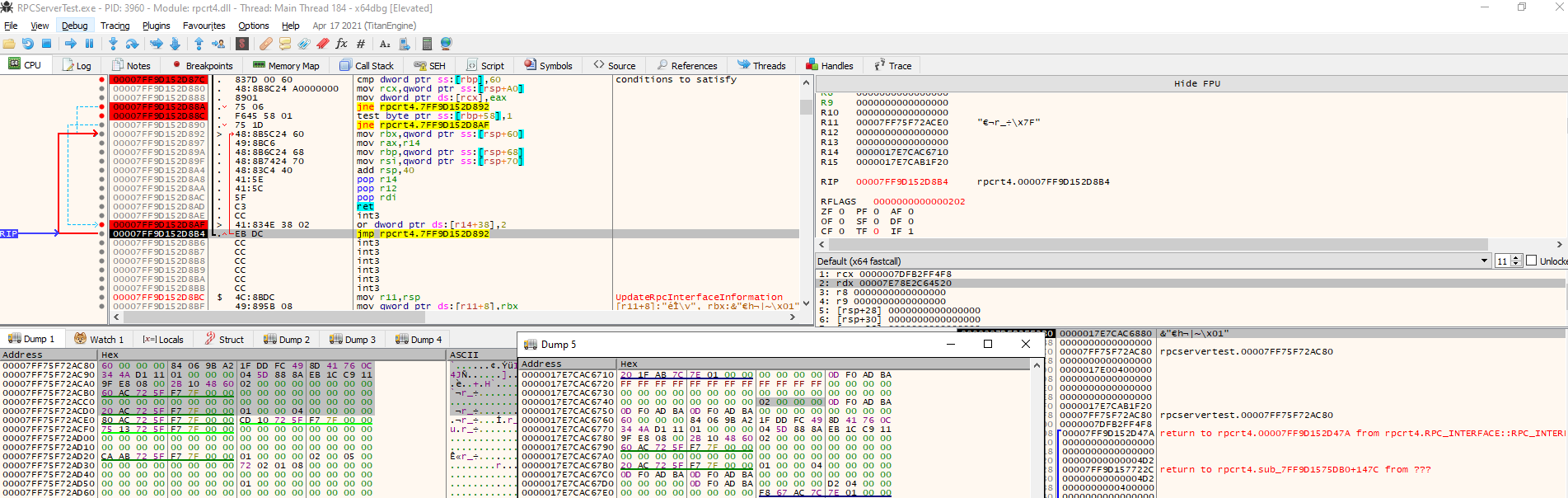Pay attention that this is not triggered by the client, in the previous screen the server just configure itself, no client connected to it. Finally in the `BeginRpcCall()` the check is satisfied.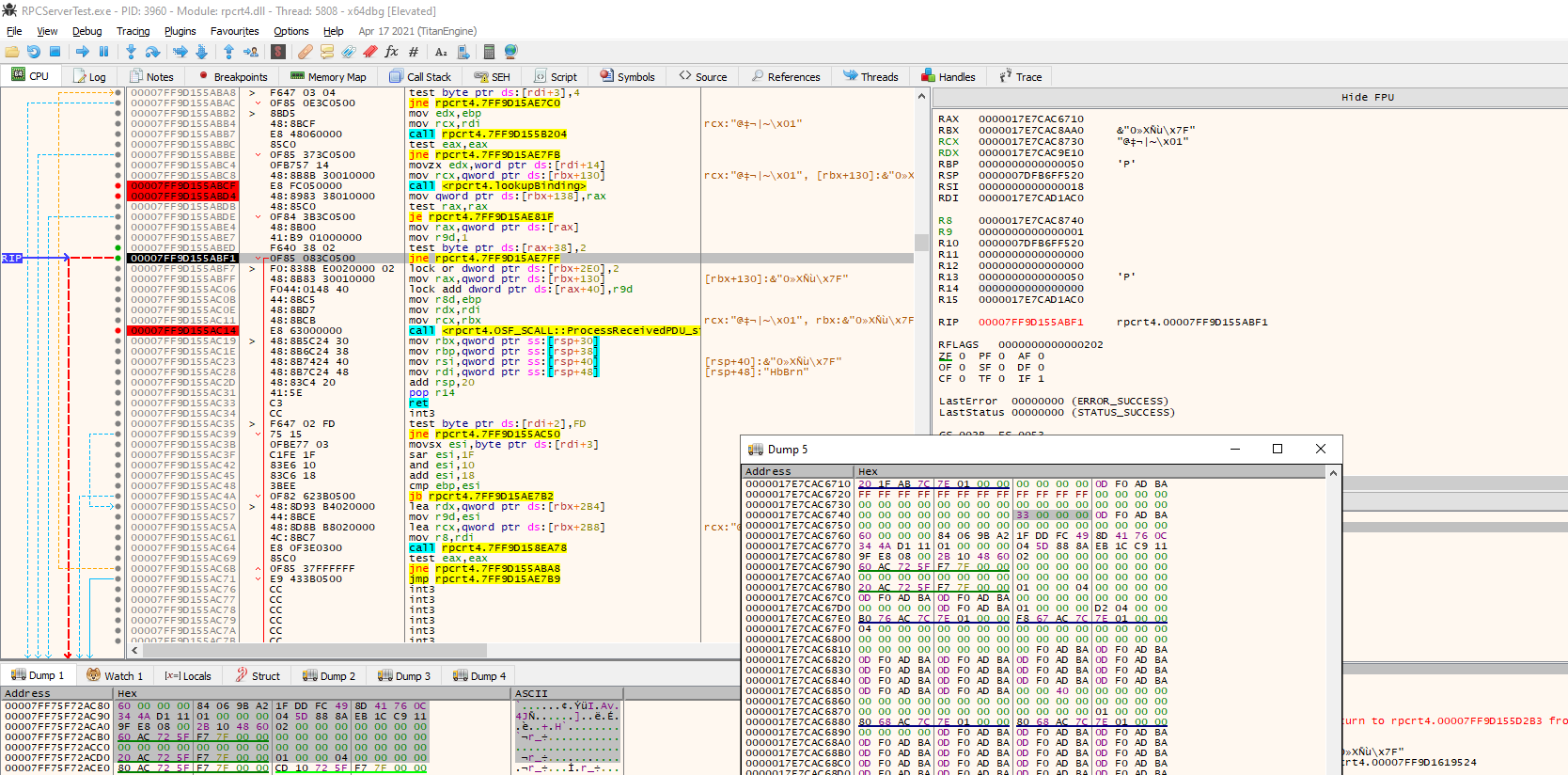Finally, the check inside the `ProcessReceivedPDU()` is satisfied, but the vulnerable code still not reached because of:

``````ulonglong OSF_SCALL::ProcessReceivedPDU (OSF_SCALL *this,dce_rpc_t *tcp_payload,uint len,int auth_fixed?)
{
...
if ((*(int *)(this + 0x244) == 0) || (*(int *)(this + 0x1cc) != 0)) { // not enter here!
...
}
...
else if (pkt_tyoe == 0) { // packet is a request
/* should not enter here  */
if (*(int *)(this + 0x214) == 0) {
...
goto _OSF_SCALL::DispatchRPCCall // that handle the request
}
//vulnerable code
}

...
}

``````

The problem now is to find under which conditions `(this + 0x214) is set`. I already saw that in the `ActivateCall()` routine called every time an RPC communication starts `*(this + 0x214) is set to 0`. For this, for sure on first call that value cannot be different by 0.

I decided to dig a bit in the code just to understand better how the messages are handled. The messages are processed according to the packet type defined in the DCERPC field,Reference, in the routine `void OSF_SCONNECTION::ProcessReceiveComplete()`.

``````void OSF_SCONNECTION::ProcessReceiveComplete{

if (pkt_type == 0) { // packet is a request
if (*(int *)(this + 3) == 0) { // not investigated
if (*(int *)((longlong)this + 0x17c) < (int)uVar6) { //should enter here if the call id is new, i.e. the packet refer to a new request
...
ppvVar9 = (LPVOID *)OSF_SCALL::OSF_SCALL(puVar11,(longlong)this,(long *)pdVar18); // maybe instantiate the object to represent the call request
...
iVar4 = OSF_SCALL::BeginRpcCall((longlong *)ppvVar9,tcp_received,ppOVar20);
...
}
else{
ppvVar9 = (LPVOID *)FindCall((OSF_SCONNECTION *)this,uVar6); // the call id has already view in the communication there should be an object, FIND IT!
if (ppvVar9 == (LPVOID *)0x0) { // not found this is a new call id request, should have first fragment set and not reuse already used call ids?
if (((int)uVar6 < *(int *)((longlong)this + 0x17c)) ||
((tcp_received->pkt_flags & 1U) == 0)) { // should not be
uVar6 = *(int *)((longlong)this + 0x17c) - uVar6;
goto reach_fault?;
}
goto LAB_18009016d;
}
call_processReceivedPDU: // call id object found just process new message
...
}
}
else
{
if ((*(byte *)&tcp_received->data_repres & 0xf0) != 0x10) {
uVar6 = uVar6 >> 0x18 | (uVar6 & 0xff0000) >> 8 | (uVar6 & 0xff00) << 8 | uVar6 << 0x18;
}
if ((tcp_received->pkt_flags & 1U) == 0) {
if (*(int *)((longlong)this + 0x1c) != 0) {
uVar6 = *(int *)((longlong)this + 0x17c) - uVar6;
reach_fault?:
if (0x95 < uVar6) goto goto_SendFAULT;
goto LAB_180090122;
}
}
else if (*(int *)((longlong)this + 0x1c) != 0) { // this+0x1c could be a flag true or false that says if the call id is new or not
*(undefined4 *)((longlong)this + 0x1c) = 0;
*(uint *)((longlong)this + 0x17c) = uVar6;
*(uint *)(this[0xf] + 0x1c8) = uVar6;
iVar4 = OSF_SCALL::BeginRpcCall((longlong *)this[0xf],tcp_received,ppOVar20);
goto LAB_18003d2b5;
}
LAB_18008ffc1:
iVar4 = (int)uVar8;
}
...
}
...
if (tcp_received->pkt_type == '\x0b') { // Executed when the client send a BIND request
if (this != 0) {
uVar3 = 0;
goto LAB_18008ff47;
}
iVar4 = AssociationRequested((OSF_SCONNECTION *)this,tcp_received,uVar13,uVar6);
}
...
}
``````

`BeginRpcCall()` ends in calling `ProcessReceivedPDU()` that is the primary function doing the packet parse job. `ProcessReceivedPDU()` when arrive the first and last fragment request:

``````ulonglong OSF_SCALL::ProcessReceivedPDU (OSF_SCALL *this,dce_rpc_t *tcp_payload,uint len,int auth_fixed?){
...
*(uint *)(this + 0x1d8) = local_res8; // set the fragment len in the object
*(char **)pdVar1 = tcp_payload->data;
dispatchRPCCall:
/* if last frag set with first frag */
*(undefined4 *)(this + 0x21c) = 3;
if (((byte)this[0x2e0] & 4) != 0) {
LOCK();
*(uint *)(*(longlong *)(this + 0x130) + 0x1ac) =
*(uint *)(*(longlong *)(this + 0x130) + 0x1ac) & 0xfffffffd;
*(uint *)(this + 0x2e0) = *(uint *)(this + 0x2e0) & 0xfffffffb;
}
plVar13 = (longlong *)((ulonglong)tcp_payload & 0xffffffffffffff00 | (ulonglong)pkt_tyoe);
uVar9 = DispatchRPCCall((longlong *)this,plVar13,puVar17); // process the remote call
return uVar9;
...
}
``````

`ProcessReceivedPDU()` when arrives the first fragment fragment request with last fragment flag unset:

``````ulonglong OSF_SCALL::ProcessReceivedPDU (OSF_SCALL *this,dce_rpc_t *tcp_payload,uint len,int auth_fixed?){
...
/* it is not a the last frag */
uVar5 = tcp_payload->alloc_hint; // client suggest to the server how many bytes it will need to handle the full request
if (uVar5 == 0) {
*(uint *)(this + 0x248) = local_res8;
uVar5 = local_res8; // set to the frag len if alloc hint is 0
}
else {
*(uint *)(this + 0x248) = uVar5;
}
puVar17 = (uint *)(ulonglong)uVar5;
/* Could not allocate more than: (this +0x138)+0x148 = 0x400000 */
if (*(uint *)(**(longlong **)(this + 0x138) + 0x148) <= uVar5 &&
uVar5 != *(uint *)(**(longlong **)(this + 0x138) + 0x148)) { // check to avoid possible integer overflow
piVar11 = local_80;
uVar18 = 0x1072;
local_80 = 3;
local_78 = uVar5;
}
*(undefined4 *)(this + 0x1d8) = 0;
pdVar14 = pdVar1;
/* allocate buffer for all the fragments data */
lVar7 = GetBufferDo((OSF_SCALL *)tcp_payload->data,(void **)pdVar1,uVar5,0,0, in_stack_ffffffffffffff60);
if (lVar7 == 0) goto do_;
fail:
pdVar14 = (dce_rpc_t *)0xe;
goto cleanupAndSendFault;
...
}

long __thiscall
OSF_SCALL::GetBufferDo
(OSF_SCALL *this,void **param_1,uint len,int param_3,uint param_4,ulong param_5)

{
long lVar1;
OSF_SCALL *pOVar2;
OSF_SCALL *dst;
ulonglong uVar3;
OSF_SCALL *local_res8;

local_res8 = this;
lVar1 = OSF_SCONNECTION::TransGetBuffer((OSF_SCONNECTION *)this,&local_res8,len + 0x18);
if (lVar1 == 0) {
if (param_3 == 0) {
*param_1 = local_res8 + 0x18;
}
else {
uVar3 = (ulonglong)param_4;
dst = local_res8 + 0x18;
pOVar2 = dst;
memcpy(dst,*param_1,param_4);
if ((longlong)*param_1 - 0x18U != 0) {
BCACHE::Free((ulonglong)pOVar2,(longlong)*param_1 - 0x18U,uVar3);
}
*param_1 = dst;
}
lVar1 = 0;
}
else {
lVar1 = 0xe;
}
return lVar1;
}
``````

If the fragment received is the first but not the last then a new memory is allocated using `GetBufferDo()` routine. It’s interesting the check on the allocation_hint/fragment len that cannot be greater or equal to 0x400000, this is very important because of the strange implementation of `GetBufferDo()` that allocate `len + 0x18` bytes.

If the packet is not the first fragment and not the last fragment:

``````ulonglong OSF_SCALL::ProcessReceivedPDU (OSF_SCALL *this,dce_rpc_t *tcp_payload,uint len,int auth_fixed?){
...
/* should enter this branch */
if (*(void **)pdVar1 != (void *)0x0) {
do_:
uVar5 = local_res8;
if ((*(int *)(this + 0x244) == 0) || (*(int *)(this + 0x1cc) != 0)) { // !RPC_INTERFACE_HAS_PIPES
if ((*(int *)(this + 0x214) == 0) || (*(int *)(this + 0x1cc) != 0)) {
/* fragment len + current copied */
uVar5 = local_res8 + *(uint *)(this + 0x1d8);
/* alloc_hint <= fragment length  */
if (*(uint *)(this + 0x248) <= uVar5 && uVar5 != *(uint *)(this + 0x248)) {
if (*(int *)(this + 0x1cc) != 0) goto LAB_18008ea09;
*(uint *)(this + 0x248) = uVar5;
/* overflow check 0x40000  */
puVar17 = (uint *)(**(OSF_SCALL ***)(this + 0x138) + 0x148);
if (*puVar17 <= uVar5 && uVar5 != *puVar17) {
piVar11 = local_50;
uVar18 = 0x1073;
local_50 = 3;
local_48 = uVar5;
}
// reallocate because fragment len is greater than the alloc hint used during first fragment!
lVar7 = GetBufferDo(\**(OSF_SCALL ***)(this + 0x138),(void **)pdVar1,uVar5,1,
*(uint *)(this + 0x1d8),in_stack_ffffffffffffff60);
if (lVar7 != 0) goto fail;
}
uVar5 = local_res8;
puVar17 = (uint *)(ulonglong)local_res8;
//copy fragment data into the alloced buffer
memcpy((void *)((longlong)*(void **)pdVar1 + (ulonglong)*(uint *)(this + 0x1d8)),tcp_payload->data,local_res8);
*(uint *)(this + 0x1d8) = *(int *)(this + 0x1d8) + uVar5;
if ((pkt_flags & 2) == 0) {
return 0; // if it is not the last fragment return 0
}
goto dispatchRPCCall; // dispatch the request only if it is the last fragment
}
}
else if (pkt_tyoe == 0) {
/* here if the packet type is 0, i.e. request */
if (*(int *)(this + 0x214) == 0) {
...
uVar15 = GetBufferDo(\**(OSF_SCALL ***)(this + 0x138),(void **)pdVar1,uVar15,1, *(uint *)(this + 0x1d8),in_stack_ffffffffffffff60);
pdVar14 = (dce_rpc_t *)(ulonglong)uVar15;
if (uVar15 != 0) goto cleanupAndSendFault;
...
memcpy((void *)((ulonglong)*(uint *)(this + 0x1d8) + *(longlong *)(this + 0xe0)), tcp_payload->data,uVar5);
...
*(uint *)(this + 0x1d8) = *(int *)(this + 0x1d8) + uVar5;
(\**(code \**)(**(longlong **)(this + 0x130) + 0x40))();
if (*(int *)(this + 0x1d8) != *(int *)(this + 0x248)) {
return 0;
}
if ((pkt_flags & 2) == 0) {
*(undefined4 *)(this + 0x230) = 0;
}
else {
*(undefined4 *)(this + 0x21c) = 3;
if (((byte)this[0x2e0] & 4) != 0) {
LOCK();
*(uint *)(*(longlong *)(this + 0x130) + 0x1ac) =
*(uint *)(*(longlong *)(this + 0x130) + 0x1ac) & 0xfffffffd;
*(uint *)(this + 0x2e0) = *(uint *)(this + 0x2e0) & 0xfffffffb;
}
}
plVar13 = (longlong *)0x0;
goto Dispatch_rpc_call;
}
else{
...
/* store frag len */
uVar5 = local_res8;
iVar8 = QUEUE::PutOnQueue((QUEUE *)(this + 600),tcp_payload->data,local_res8);
if (iVar8 == 0) {
/* integer overflow! */
*(uint *)(this + 0x24c) = *(int *)(this + 0x24c) + uVar5;
...
}
}
``````

Basically, the middle fragments are just copied in the alloced buffer under some conditions.

The `DispatchRPC()` seems to be the dispatcher of the full request, indeed it calls then the routine to handle the request, unmarshalling parameters and executing the
procedure remotely invoked. It is interesting that:

``````undefined8 OSF_SCALL::DispatchRPCCall(longlong *param_1,longlong *param_2,undefined8 param_3)
{
if (*(int *)((longlong)param_1 + 0x1cc) < 1) {
*(undefined4 *)((longlong)param_1 + 0x214) = 1;
...
}
``````

`*(int *)((longlong)param_1 + 0x1cc` seems to be zero so ` *(undefined4 *)((longlong)param_1 + 0x214) = 1;` this instruction takes place.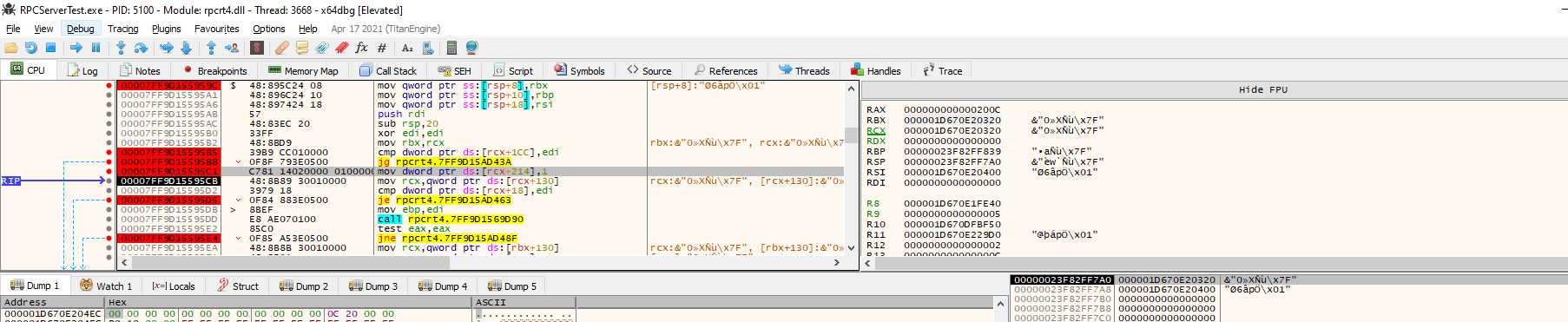It’s possible to force the `DispatchRpcCall()` and still accept fragments for the same call id? The response to this question is yes!

Looking at `ProcessReceivedPDU()` exists a path that leads to execute the DispatchRpcCall() without sending any last fragment. The constraints:

1. Server configured with RPC_INTERFACE_HAS_PIPES -> int at offset 0x58 in the rpc interface defined in the server has second bit to 1
2. Client sends a first fragment, that initiliaze the call id object
3. Client sends the second fragment without first and last flag enabled, this ends to call `DispatchRpcCall()` that sets `param_1 + 0x214 to 1`.
4. Client alloc hint sent in the first fragment must be equal to the copied buffer len at the end of second fragment.

This will lead the code to enter `DispatchRpcCall()` and make nexts client’s fragments to be handled by the buggy code.

``````ulonglong OSF_SCALL::ProcessReceivedPDU (OSF_SCALL *this,dce_rpc_t *tcp_payload,uint len,int auth_fixed?){
...
else if (pkt_tyoe == 0) {
/* here if the packet type is 0, i.e. request */
if (*(int *)(this + 0x214) == 0) {
puVar17 = (uint *)(ulonglong)local_res8;
uVar15 = *(uint *)(this + 0x1d8) + local_res8;
...
if (*(int *)(this + 0x1d8) != *(int *)(this + 0x248)) { // cheat on this to make fragments appear as the last!
// 0x1d8 contain already copied bytes until this request!
// 0x248 contains the alloc hint!
return 0;
}
if ((pkt_flags & 2) == 0) {
*(undefined4 *)(this + 0x230) = 0;
}
else {
*(undefined4 *)(this + 0x21c) = 3;
if (((byte)this[0x2e0] & 4) != 0) {
LOCK();
*(uint *)(*(longlong *)(this + 0x130) + 0x1ac) =
*(uint *)(*(longlong *)(this + 0x130) + 0x1ac) & 0xfffffffd;
*(uint *)(this + 0x2e0) = *(uint *)(this + 0x2e0) & 0xfffffffb;
}
}
plVar13 = (longlong *)0x0;
goto Dispatch_rpc_call;
...
}
``````

At this point, it’s possible to write a PoC following the previous constraints, the PoC has been tested against my RPC Server using `ncacn_np` protocol.

With the poc seems to be impossible to trigger the vulnerability due some checks in `ProcessReceivedPDU()`: Basically the check, that makes impossible to overflow the integer, is executed on the queue messages size.

``````OSF_SCALL::ProcessReceivedPDU()
{
...
iVar8 = QUEUE::PutOnQueue((QUEUE *)(this + 600),tcp_payload->data,local_res8);
if (iVar8 == 0) {
/* integer overflow! */
*(uint *)(this + 0x24c) = *(int *)(this + 0x24c) + uVar5;
if (((pkt_flags & 2) != 0) &&
(*(undefined4 *)(this + 0x21c) = 3, ((byte)this[0x2e0] & 4) != 0)) {
LOCK();
*(uint *)(*(longlong *)(this + 0x130) + 0x1ac) =
*(uint *)(*(longlong *)(this + 0x130) + 0x1ac) & 0xfffffffd;
*(uint *)(this + 0x2e0) = *(uint *)(this + 0x2e0) & 0xfffffffb;
}
if (*(longlong *)(this + 0x18) != 0) {
if ((*(uint *)(this + 0x250) == 0) ||
((*(uint *)(this + 0x24c) < *(uint *)(this + 0x250) &&
(*(int *)(this + 0x21c) != 3)))) {
if ((3 < *(int *)(this + 0x264)) &&
((*(int *)(*(longlong *)(this + 0x130) + 0x18) != 0 &&
(uVar9 = 0, *(int *)(this + 0x21c) != 3)))) {
*(undefined4 *)(this + 0x2ac) = 1;
uVar9 = uVar19; // critical instruction that makes processreceivepdu return 1
}
}
else {
*(undefined4 *)(this + 0x250) = 0;
}
RtlLeaveCriticalSection(pOVar3);
return uVar9;
}
if (((3 < *(int *)(this + 0x264)) &&
(*(int *)(*(longlong *)(this + 0x130) + 0x18) != 0)) &&
(*(int *)(this + 0x21c) != 3)) {
*(undefined4 *)(this + 0x2ac) = 1;
uVar9 = uVar19; // critical instruction that makes processreceivepdu return 1; My code entered heres
}
RtlLeaveCriticalSection(pOVar3);
SetEvent(*(HANDLE *)(this + 0x2c0));
return uVar9; //
}
...
``````

When `ProcessReceivedPDU()` returns 1, it stops to listen for new messages so the client cannot trigger vulnerability anymore.

``````LAB_18008ffc1:
iVar4 = (int)uVar8;
}
LAB_18003d2b5:
if (iVar4 == 0) {
LAB_18003d3c3:
(**(code **)(*this + 0x38))(); -> OSF_SCONNECTION::TransAsyncReceive() continues to read from the descriptor new packets
}
REFERENCED_OBJECT::RemoveReference(this);
goto LAB_18003d2c5;
}
``````

In order to not enter the critical paths that lead the server stopping listen for new packets, in the same connection of course, it’s required that one of these should not be satisfied:

``````3 < *(int *)(this + 0x264) // number of queued packets
*(int *)(*(longlong *)(this + 0x130) + 0x18) != 0 // don't know
*(int *)(this + 0x21c) != 3  // can be unsatisfied on the packet with last fragmente flag enabled

*(this + 0x21c)  is set to 3 if the packets has the last fragment enabled.
``````

The number of queued packets is incremented on a `PutOnQueue()` and decremented on a `TakeOffQueue()`, at most the queue could contain 4 packets. To me this was so strange because the application was multithread but looked like a monothread just because I did’t see any `TakeOffQueue()` called during my experiments.

``````int __thiscall QUEUE::PutOnQueue(QUEUE *this,void *param_1,uint param_2)
{
...
*(int *)(this + 0xc) = iVar6 + 1;
**(void ***)this = param_1;
*(uint *)(*(longlong *)this + 8) = param_2;
return 0;
}

undefined8 QUEUE::TakeOffQueue(longlong *param_1,undefined4 *param_2)
{
int iVar1;

iVar1 = *(int *)((longlong)param_1 + 0xc);
if (iVar1 == 0) {
return 0;
}
*(int *)((longlong)param_1 + 0xc) = iVar1 + -1;
*param_2 = *(undefined4 *)(*param_1 + -8 + (longlong)iVar1 * 0x10);
return *(undefined8 *)(*param_1 + (longlong)*(int *)((longlong)param_1 + 0xc) * 0x10);
}
``````

In general my thought on the server status was the following:

1. A Thread running somewhere in the `DispatcRpcCall()` after the packet that forced to call `DispatchRpcCall()` has been received, i.e. constrants number 3.
2. A Thread continuing to read from the medium, did not know from.

So, I inspected better `DispatcRpcCall()`.

``````undefined8 OSF_SCALL::DispatchRPCCall(longlong *param_1,longlong *param_2,undefined8 param_3)
{
...
uVar3 = RpcpConvertToLongRunning(this,param_2,param_3);
bVar7 = (int)uVar3 != 0;
if (bVar7) {
uVar1 = 0xe;
}
else {
this = (longlong *)param_1[0x26];
uVar1 =  OSF_SCONNECTION::TransAsyncReceive(); // this should wake up a thread to handle new incoming packet
}
if (uVar1 == 0) {
pOVar6 = (OSF_SCONNECTION *)param_1[0x26];
DispatchHelper(param_1);
if (*(int *)(pOVar6 + 0x18) == 0) { // this is the (this + 0x130) + 0x18)
OSF_SCONNECTION::DispatchQueuedCalls(pOVar6);
}
pcVar4 = OSF_SCALL::RemoveReference(); // calling this means that the connection is over!
}
else {
uVar3 = param_1[0x1c] - 0x18;
if (uVar3 != 0) {
BCACHE::Free((ulonglong)this,uVar3,param_3);
}
if (bVar7) {
CleanupCallAndSendFault(param_1,(ulonglong)uVar1,0);
}
else {
CleanupCall(param_1,uVar3,param_3);
}
pOVar6 = (OSF_SCONNECTION *)param_1[0x26];
if (*(int *)(pOVar6 + 0x18) == 0) {
OSF_SCONNECTION::AbortQueuedCalls(pOVar6);
pOVar6 = (OSF_SCONNECTION *)param_1[0x26];
}
...
}

int OSF_SCONNECTION::TransAsyncReceive(longlong *param_1,undefined8 param_2,undefined8 param_3)

{
int iVar1;

if (*(int *)(param_1 + 5) == 0) {
/* CO_Recv */
iVar1 = CO_Recv(); // return true if a packet has been read
if (iVar1 != 0) {
if ((*(int *)(param_1 + 3) != 0) && (*(int *)((longlong)param_1 + 0x1c) == 0)) {
}
*(undefined4 *)(param_1 + 5) = 1;
AbortConnection(param_1);
}
}
else {
AbortConnection(param_1);
iVar1 = -0x3ffdeff8;
}
return iVar1;
}

ulonglong CO_Recv(PSLIST_HEADER param_1,undefined8 param_2,SIZE_T param_3)

{
uint uVar1;
ulonglong uVar2;

if ((*(int *)&param_1.field_0x8 != 0) &&
(*(int *)&param_1.field_0x8 == *(int *)&param_1.field_0x4)) {
uVar2 = (ulonglong)uVar1;
if (uVar1 != 0) {
uVar2 = 0xc0021009;
}
return uVar2;
}
uVar2 = CO_SubmitRead(param_1,param_2,param_3); // ends in calling NMP_CONNECTION::Receive
return uVar2;
}
``````

According to my breakpoints, `TransAsyncReceive()` is executed and this led the server in waiting for a new packet after the packet that led to `DispatchRpcCall()`. If it, `TransAsyncReceive()`, returns 0 then the packet should has been read and handled by another thread, indeed it proceds calling `DispatchHelper()`. At this point, I imagined two threads:

1. A thread started from `TransAsyncReceive()` that handle new packet and lead to enter in the `ProcessReceivedPDU()` continues to read until it not returns 1.
2. A Thread that enters in `DispatchHelper()`.

So, I started to track the memory at `(this + 0x130) + 0x18` to identify where it’s written to 1. Because if it is not 0 then the condition that lead `ProcessReceivedPDU()` returning 1 is not satisfied anymore then it continue to read and because if it is 0 then a `TakeOffQueue()` is executed from `DispatchRpcCall()`.

``````undefined8 OSF_SCALL::DispatchRPCCall(longlong *param_1,longlong *param_2,undefined8 param_3)
{
...
if (*(int *)(pOVar6 + 0x18) == 0) {
OSF_SCONNECTION::DispatchQueuedCalls(pOVar6);
}
...
}

void __thiscall OSF_SCONNECTION::DispatchQueuedCalls(OSF_SCONNECTION *this)
{
longlong *plVar1;
undefined4 local_res8 ;

while( true ) {
RtlEnterCriticalSection(this + 0x118);
plVar1 = (longlong *)QUEUE::TakeOffQueue((longlong *)(this + 0x1b8),local_res8); // decrement no. queued messaeges
if (plVar1 == (longlong *)0x0) break;
RtlLeaveCriticalSection();
OSF_SCALL::DispatchHelper(plVar1);
...
}
...
return;
}
``````

`(this + 0x130) + 0x18` is set to 1, during the association request at offset `rpcrt4.dll+0x38b99`, just if the packet flag multiplexed is not set.

``````void OSF_SCONNECTION::AssociationRequested
(OSF_SCONNECTION *param_1,dce_rpc_t *tcp_received,uint param_3,int param_4)
{

if ((tcp_received->pkt_flags & 0x10U) == 0) {
*(undefined4 *)(param_1 + 0x18) = 1;
*(byte *)((longlong)pppppvVar21 + 3) = *(byte *)((longlong)pppppvVar21 + 3) | 3;
}
}
``````

So, setting the RPC_HAS_PIPE in the server interface and specifying MULTIPLEX flag during the connection, it is under client control, will lead the code into the vulnerable point. At this point the debugger enters in the `OSF_SCALL::GetCoalescedBuffer()` that is another vulnerable call.

The code enter here from this stack trace:

``````rpcrt4.dll+0xb404d in OSF_SCALL::Receive()
rpcrt4.dll+0x4b297 in RPC_STATUS I_RpcReceive()
rpcrt4.dll+0x82422 in NdrpServerInit()
rpcrt4.dll+0x1c35e in NdrStubCall2()
NdrServerCall2()
rpcrt4.dll+0x57832 in DispatchToStubInCNoAvrf()
rpcrt4.dll+0x39e00 in RPC_INTERFACE::DispatchToStubWorker()
rpcrt4.dll+0x39753 in DispatchToStub()
rpcrt4.dll+0x3a2bc in OSF_SCALL::DispatchHelper()
DispatchRPCCall()
``````

So using this PoC we enter every n packets in the coalesced buffer triggering the second integer overflow.

The routine `OSF_SCALL::GetCoalescedBuffer()` is reached just because the server has RPC_HAS_PIPE configured. Analyzing the stack trace from the last function before OSF_SCALL::GetCoalescedBuffer() I searched some constraints I satisfied that led me to the vulnerable code.

``````long __thiscall OSF_SCALL::Receive(OSF_SCALL *this,_RPC_MESSAGE *param_1,uint param_2)
{
...
while (iVar3 = *(int *)(this + 0x21c), iVar3 != 1) { // this+ 0x21c seems not set to 1 in ProcessReceivedPDU, did not found where it is

if (iVar3 == 2) {
return *(long *)(this + 0x20);
}
if (iVar3 == 3) { // it is equal to 3 on the last frag packet, from ProcessReceivedPDU
lVar2 = GetCoalescedBuffer(this,param_1,iVar4);
return lVar2; // last frag received -> no more packets will be waited!
}
// if the total queued packets length is less or equal to the allocation hint received than wait for new data
if (*(uint *)(this + 0x24c) <= (uint)*(ushort *)(*(longlong *)(this + 0x130) + 0xb8) {
EVENT::Wait((EVENT *)(this + 0x2c0),-1); // wait for other packets
}
else {
lVar2 = GetCoalescedBuffer(this,param_1,iVar4); // run coalesced buffer
if (lVar2 != 0) { // error
return lVar2;
}
...
}
...
}
...
}

// (uint)*(ushort *)(*(longlong *)(this + 0x130) + 0xb8 is set during the association request
void OSF_SCONNECTION::AssociationRequested
(OSF_SCONNECTION *param_1,dce_rpc_t *tcp_received,uint param_3,int param_4)
{
...
uVar18 = *(ushort *)((longlong)&tcp_received->alloc_hint + 2);
if ((*(byte *)&tcp_received->data_repres & 0xf0) != 0x10) {
uVar26 = *(uint *)&tcp_received->cxt_id;
uVar23 = uVar23 >> 8 | uVar23 << 8;
uVar18 = uVar18 >> 8 | uVar18 << 8;
*(ushort *)((longlong)&tcp_received->alloc_hint + 2) = uVar18;
uVar26 >> 0x18 | (uVar26 & 0xff0000) >> 8 | (uVar26 & 0xff00) << 8 | uVar26 << 0x18;
*(ushort *)&tcp_received->alloc_hint = uVar23;
}
puVar24 = (undefined4 *)(ulonglong)uVar23;
uVar14 = (ushort)*(undefined4 *)(*(longlong *)(param_1 + 0x20) + 0x4c);
uVar3 = 0xffff;
if (uVar23 != 0xffff) {
uVar3 = uVar23;
}
if (uVar3 <= uVar18) {
uVar18 = uVar3;
}
if (uVar18 <= uVar14) {
uVar14 = uVar18;
}
*(ushort *)(param_1 + 0xb8) = uVar14 & 0xfff8; // set equal to the allocation hint & 0xfff8 of the first association packet!
...
}
``````

Basically from `OSF_SCALL::Receive()` the code continuosly waits for new packets until a last fragment packet is received or any error is found.

`GetCoalescedBuffer()` is called if the last fragment is received or if the total len accumulated during the `ProcessReceivedPDU()` is greater than the `alloc_hint`, for these condition every n packets it is called.

So, just increasing the fragment length in the PoC it’s possible to trigger `GetCoalescedBuffer()` without setting MULTIPLEX. Moreover, `OSF_SCALL::Receive()` is reached because `Message->RpcFlags & 0x8000 == 0`

``````RPC_STATUS I_RpcReceive(PRPC_MESSAGE Message,uint Size)
{
...
if ((Message->RpcFlags & 0x8000) == 0) {
pcVar5 = *(code **)(*plVar7 + 0x38);
}
else {
/* get NDRServerInitializeMarshall address */
pcVar5 = *(code **)(*plVar7 + 0x48);
}
/* call coalesced buffer */
uVar2 = (*pcVar5)();
...
}

void NdrpServerInit()
{
...
if (param_5 == 0) {
if ((*(byte *)(*(ushort **)(puVar2 + 0x10) + 2) & 8) == 0) {
...

...
if ((param_2->RpcFlags & 0x1000) == 0) {  // During my tests this value was 0 each time, didnt searched when this could fail
param_2->RpcFlags = 0x4000;               // makes to call GetCoalescedBuffer()
puStackY96 = (undefined *)0x180082427;
if (exception != 0) {
/* WARNING: Subroutine does not return */
puStackY96 = &UNK_180082432;
RpcRaiseException(exception);
}
param_1->Buffer = (uchar *)param_2->Buffer;
puVar10 = (uchar *)param_2->Buffer;
param_1->BufferStart = puVar10;
param_1->BufferEnd = puVar10 + param_2->BufferLength;
param_3 = pMVar20;
}
...
}

``````

`GetCoalescedBuffer()` is used to merge queued buffers to the last received packet that has not been queued, indeed it allocates the total length needed and copy there every queued message then.

``````long __thiscall OSF_SCALL::GetCoalescedBuffer(OSF_SCALL *this,_RPC_MESSAGE *param_1,int param_2)
{
...
iVar5 = *(int *)(this + 0x24c);
if (iVar5 != 0) {
if (uVar3 != 0) {
/* integer overflow */
iVar5 = iVar5 + *(int *)(param_1 + 0x18);
}
lVar2 = OSF_SCONNECTION::TransGetBuffer((OSF_SCONNECTION *)this_00,&local_res8,iVar5 + 0x18);
if (lVar2 == 0) {
dst_00 = (void *)((longlong)local_res8 + 0x18);
dst = dst_00;
local_res8 = dst_00;
if ((uVar3 != 0) && (*(void **)(param_1 + 0x10) != (void *)0x0)) {
memcpy(dst_00,*(void **)(param_1 + 0x10),*(uint *)(param_1 + 0x18));
local_res8 = (void *)((ulonglong)*(uint *)(param_1 + 0x18) + (longlong)dst_00);
OSF_SCONNECTION::TransFreeBuffer() // free last message PDU received
...
}
while (src = (void *)QUEUE::TakeOffQueue((longlong *)(this + 600),(undefined4 *)&local_res8), src != (void *)0x0) {
// loop to consume the queue merging the queued packets
uVar4 = (ulonglong)local_res8 & 0xffffffff;
memcpy(dst,src,(uint)local_res8);
/* OSF_SCONNECTION::TransFreeBuffer  */
OSF_SCONNECTION::TransFreeBuffer() // free PDU buffer queued
dst = (void *)((longlong)dst + uVar4);
}
...
*(undefined4 *)(this + 0x24c) = 0;
...
}
``````

At this point from the new constraints found merging to the old ones it’s possible to resume the conditions that lead to vulnerable point:

1. Server configured with RPC_INTERFACE_HAS_PIPES -> integer at offset 0x58 in the rpc interface defined in the server has second bit to 1 - **Not under attacker control **
2. Client sends a first fragment, that initiliaze the call id object and satisfies the condition to call directly `DispatchRpcCall()`, sending fragment long just as the alloc hint set in this way: `(*(int *)(this + 0x1d8) != *(int *)(this + 0x248)` in `ProcessReceivedPDU()` lead to execute `DispatchRpcCall()` and so `param_1 + 0x214 is set to 1` - Under attacker control
3. Client continues to send middle fragments until: `iVar5 = iVar5 + *(int *)(param_1 + 0x18);` overflows and lead `memcpy()` to overflow the buffer - Under attacker control